Results as of EPS 2005

Menu:

 A brief document that provides the collection of up-to-date inputs to the global CKM analysis and the numerical results obtained from it. The results include: Wolfenstein parameters, UT angles, (combinations of) CKM elements, theory parameters and rare branching fractions. Detailed background information on the methodology and the treatment of experimental and theoretical uncertainties is provided in hep-ph/0406184.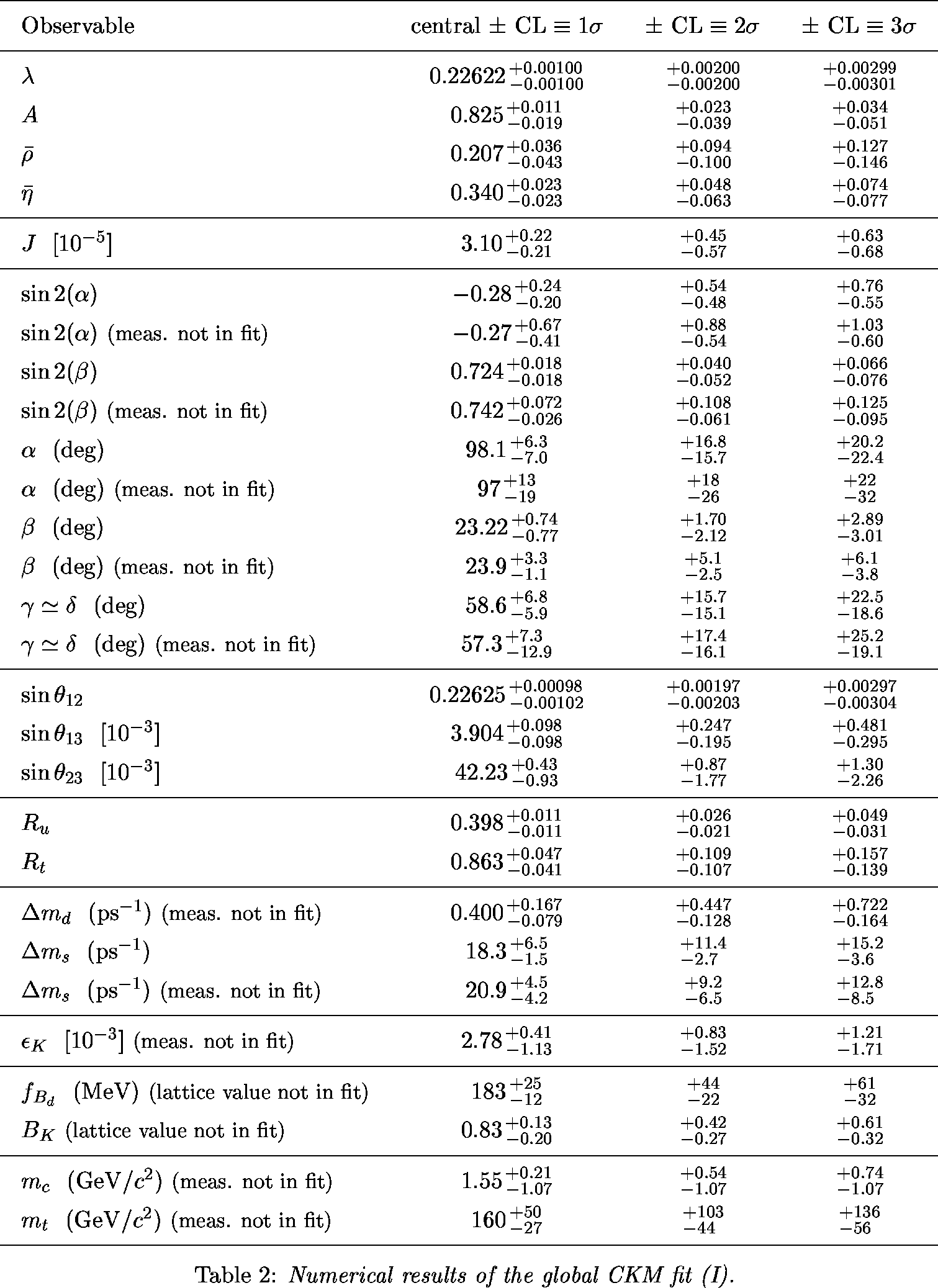ps pdf

 α,β,γ convention: φ1,φ2,φ3 convention: Constraints in the (ρ-bar,η-bar) plane including (a.o.) the most recent α/Φ2- and γ/Φ3-related inputs in the global CKM fit.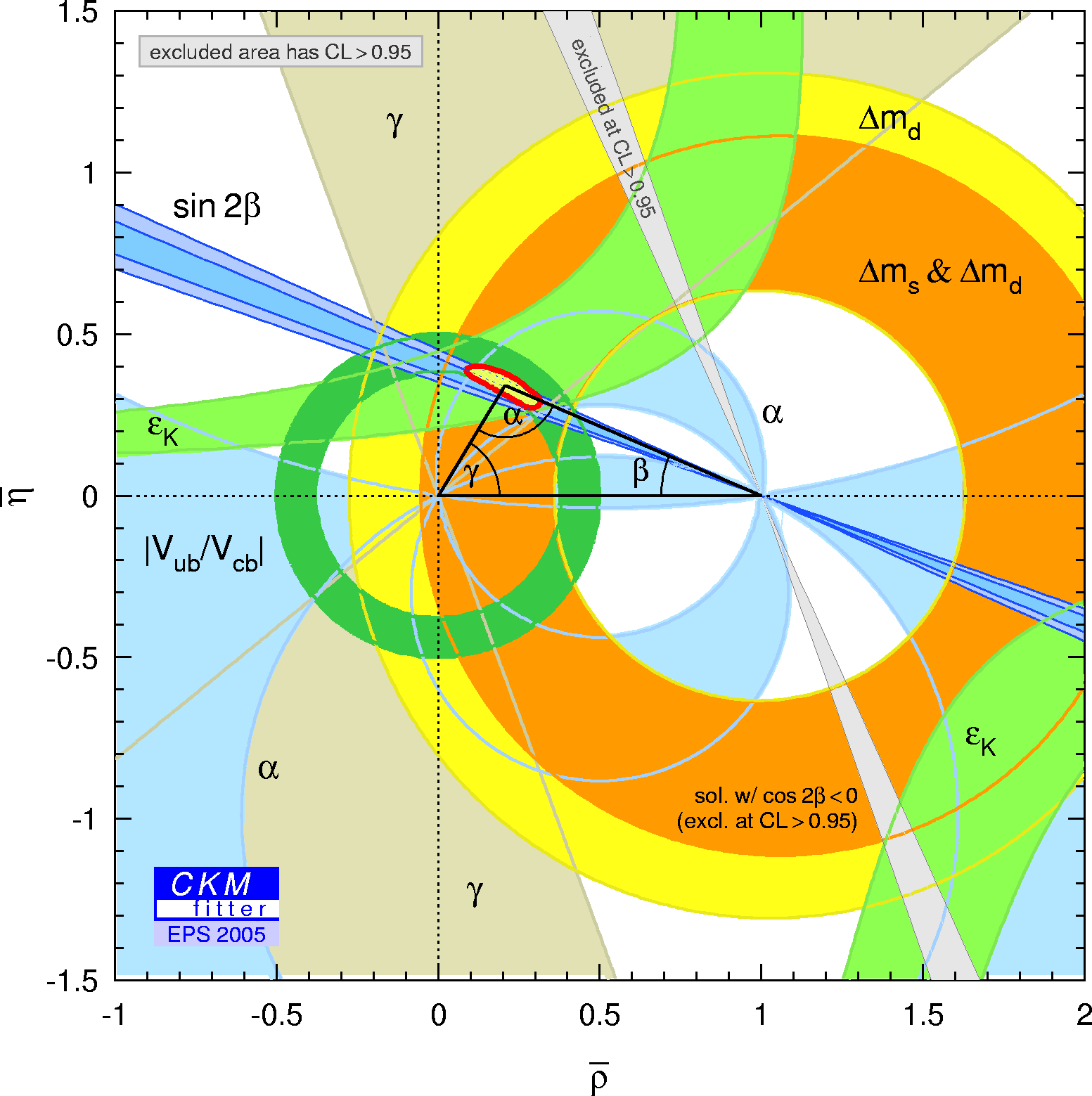eps gif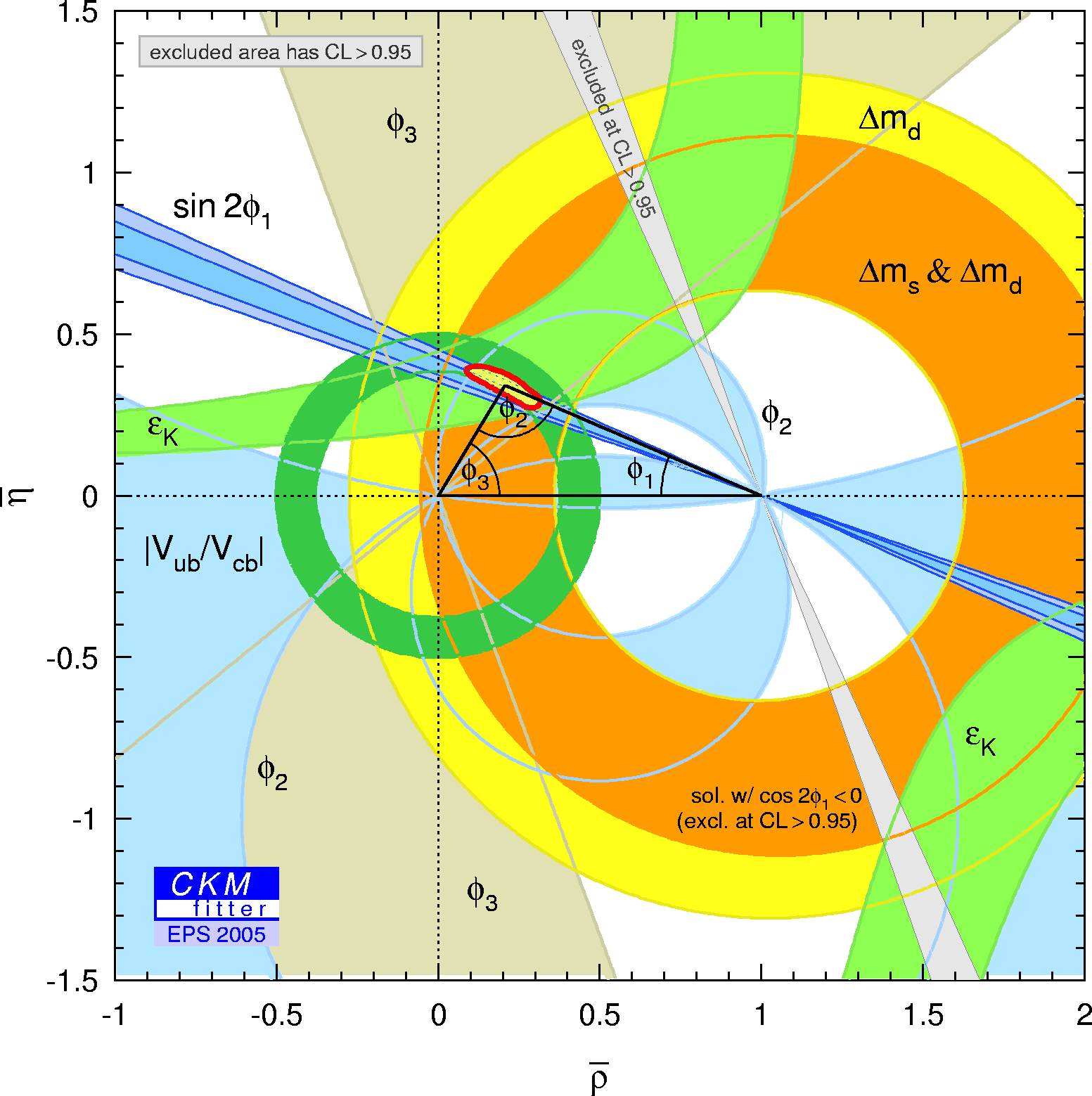eps gif Constraints in the (ρ-bar,η-bar) plane not including the angle measurements in the global fit.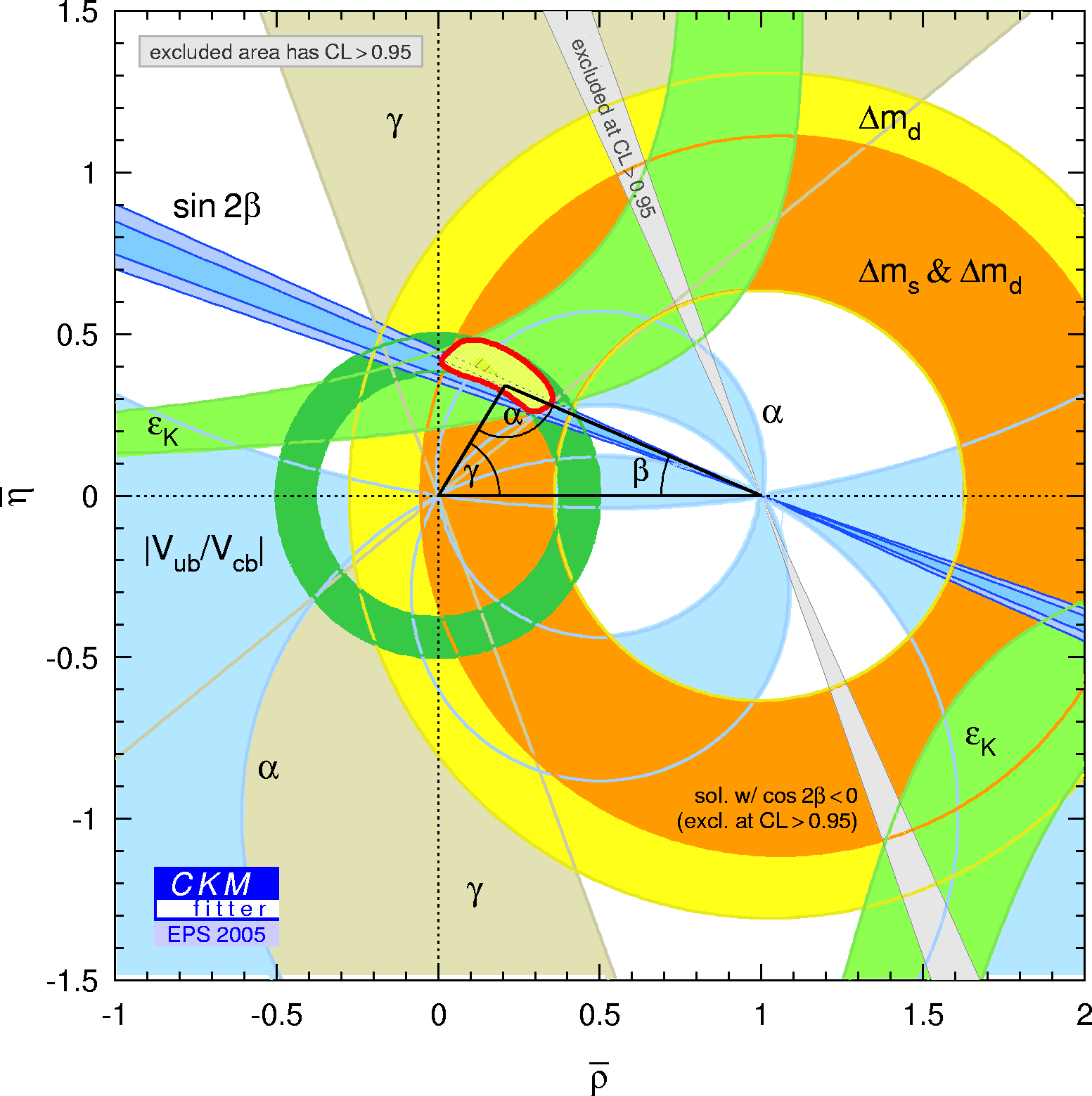eps gif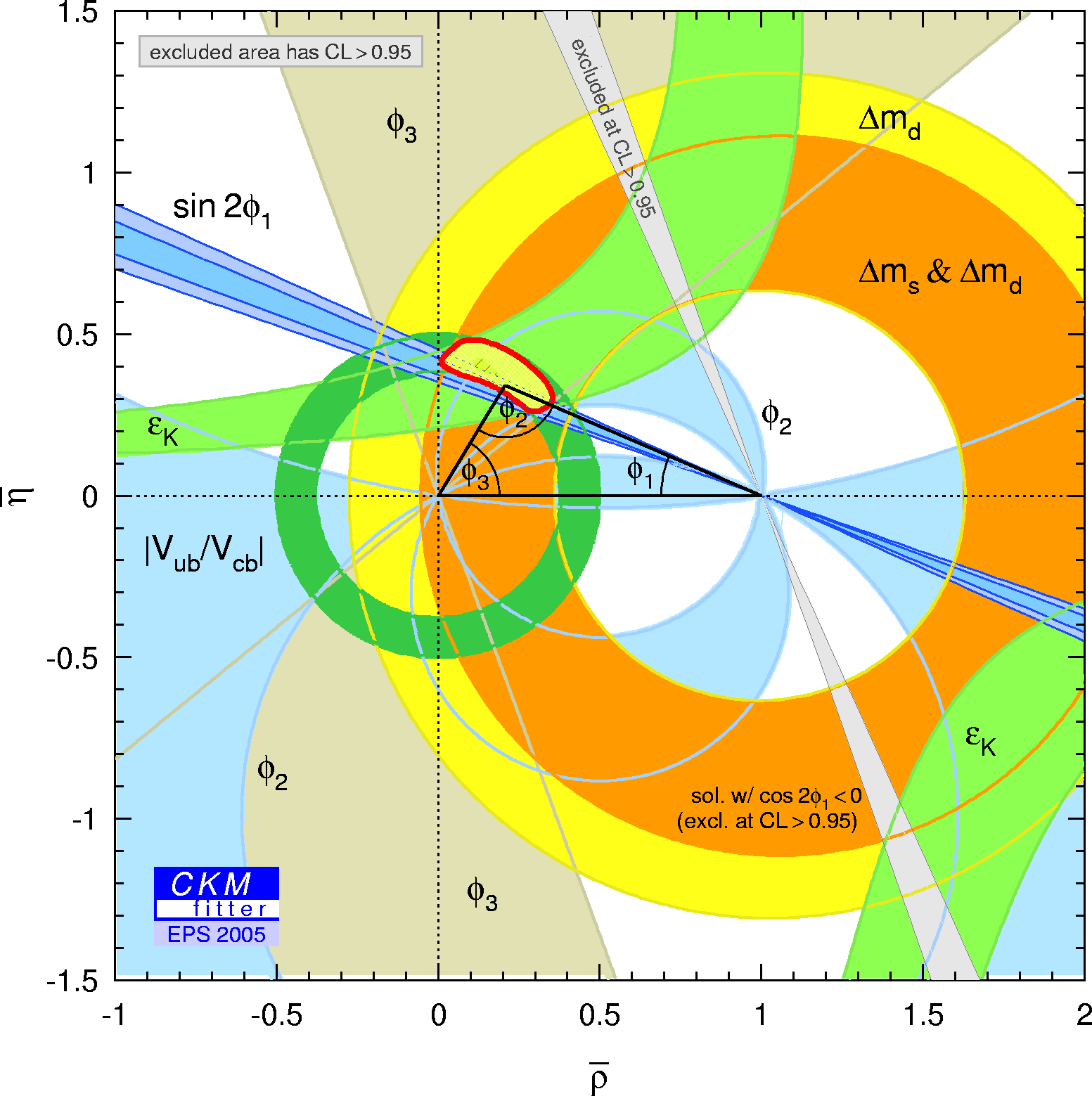eps gif Constraints in the (ρ-bar,η-bar) plane not including the α/Φ2 and γ/Φ3 measurement in the global fit. the global fit.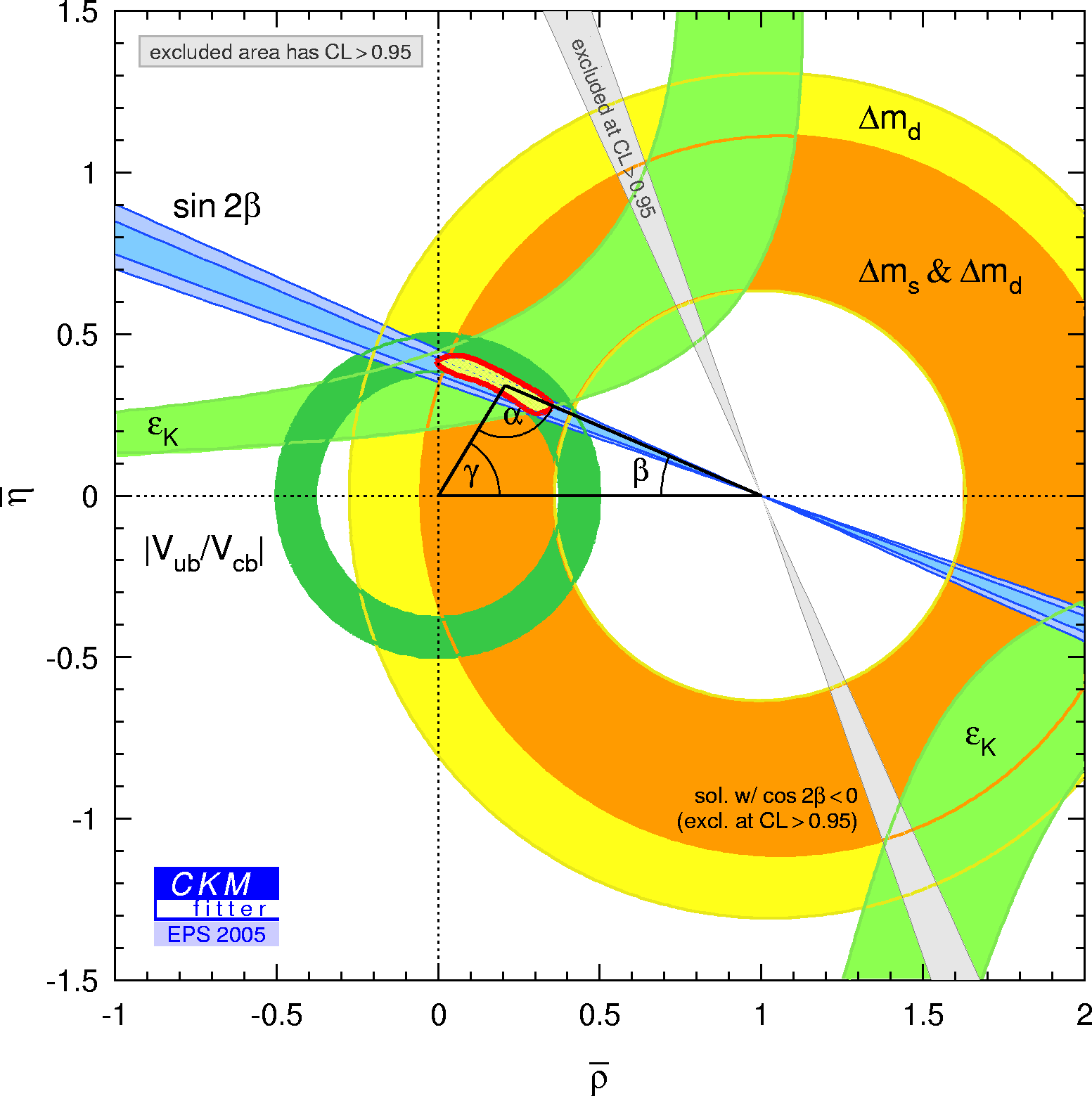eps gif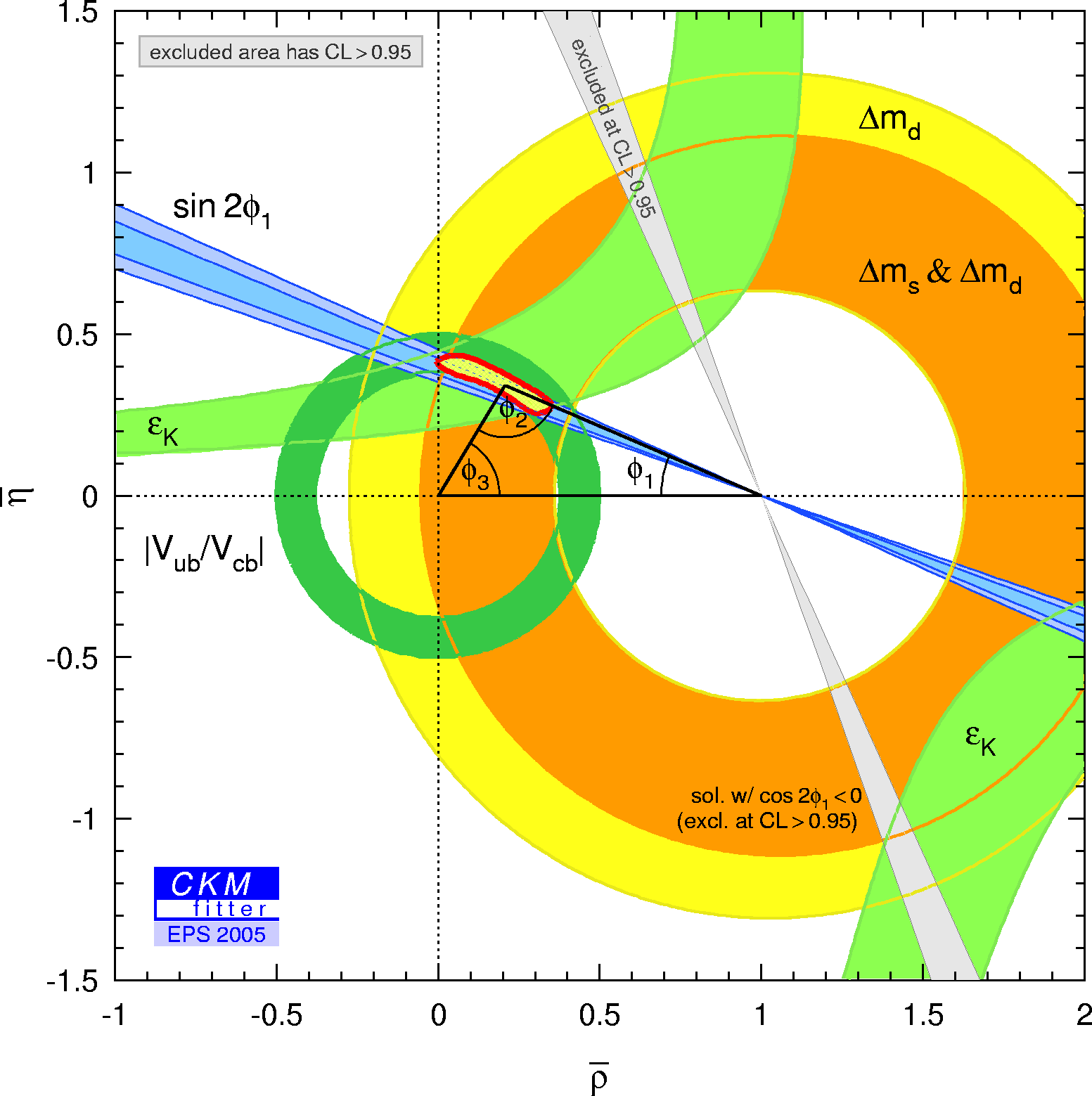eps gif Constraints in the (ρ-bar,η-bar) plane including only the angle measurements in the global fit.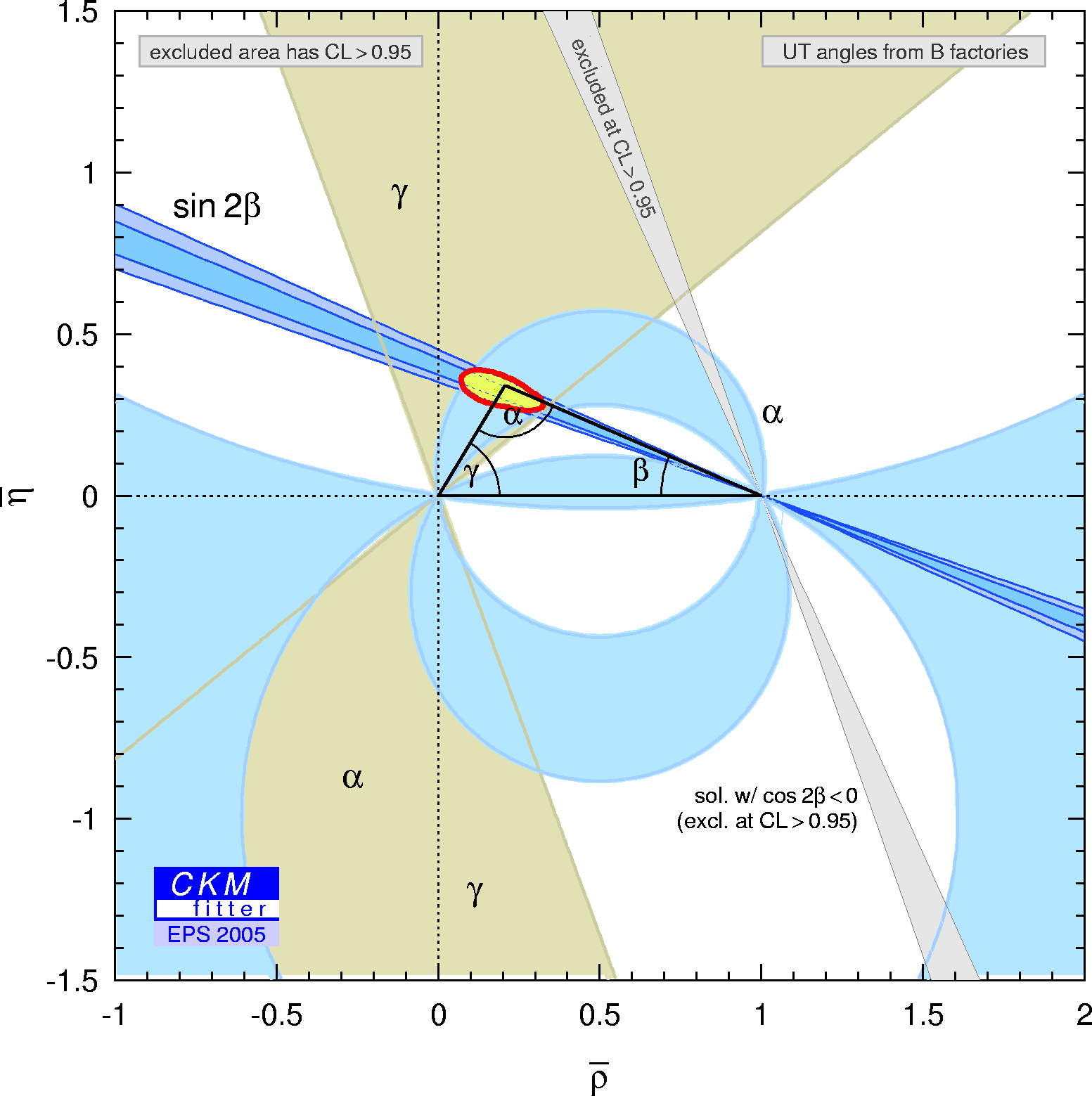eps gif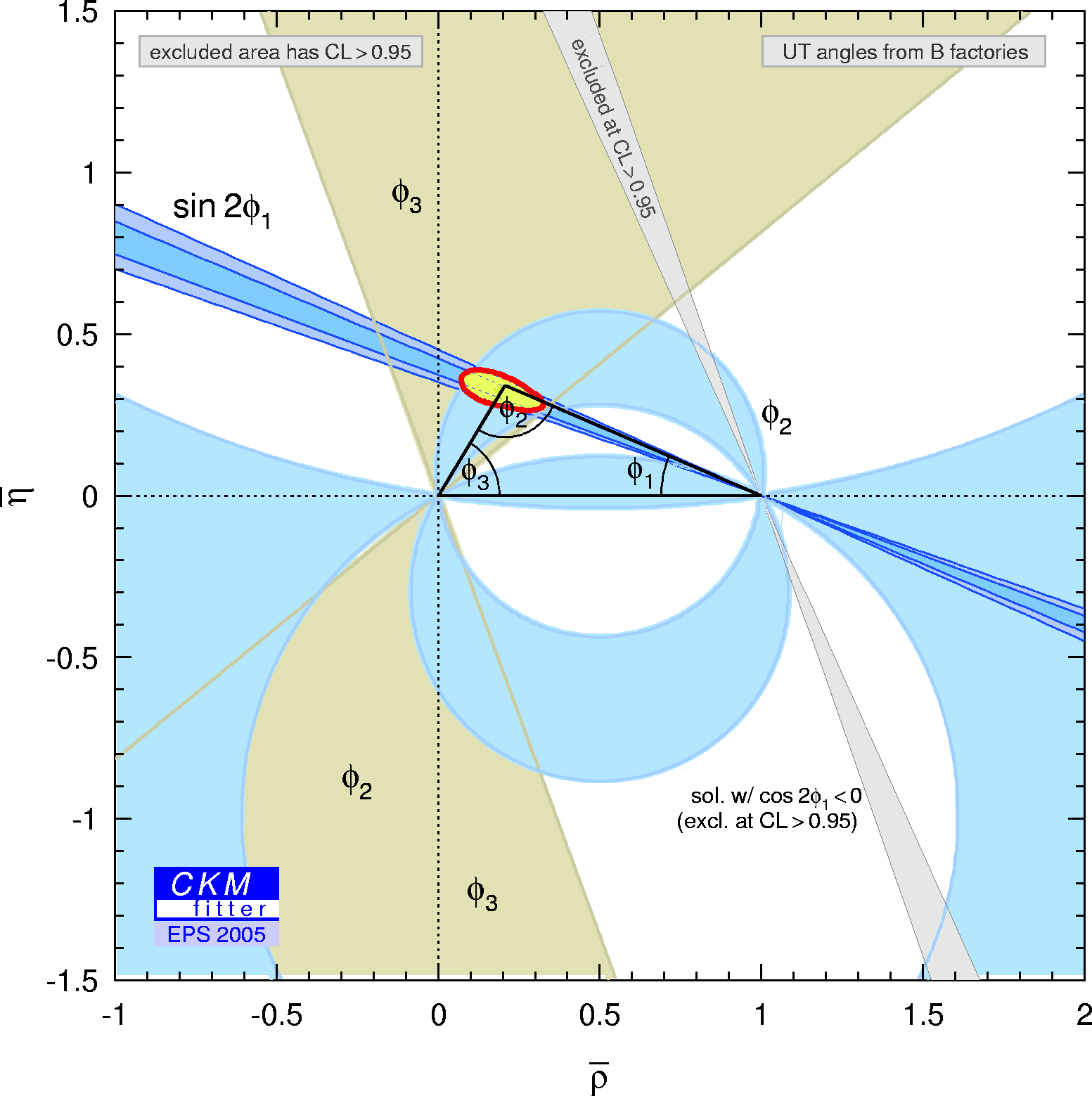eps gif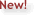"Movie" showing step-by-step the constraints in the (ρ-bar,η-bar) plane PPT FILE

 α,β,γ convention: φ1,φ2,φ3 convention: Zoomed constraints in the (ρ-bar,η-bar) plane including the most recent α/Φ2- and γ/Φ3-related inputs in the global CKM fit.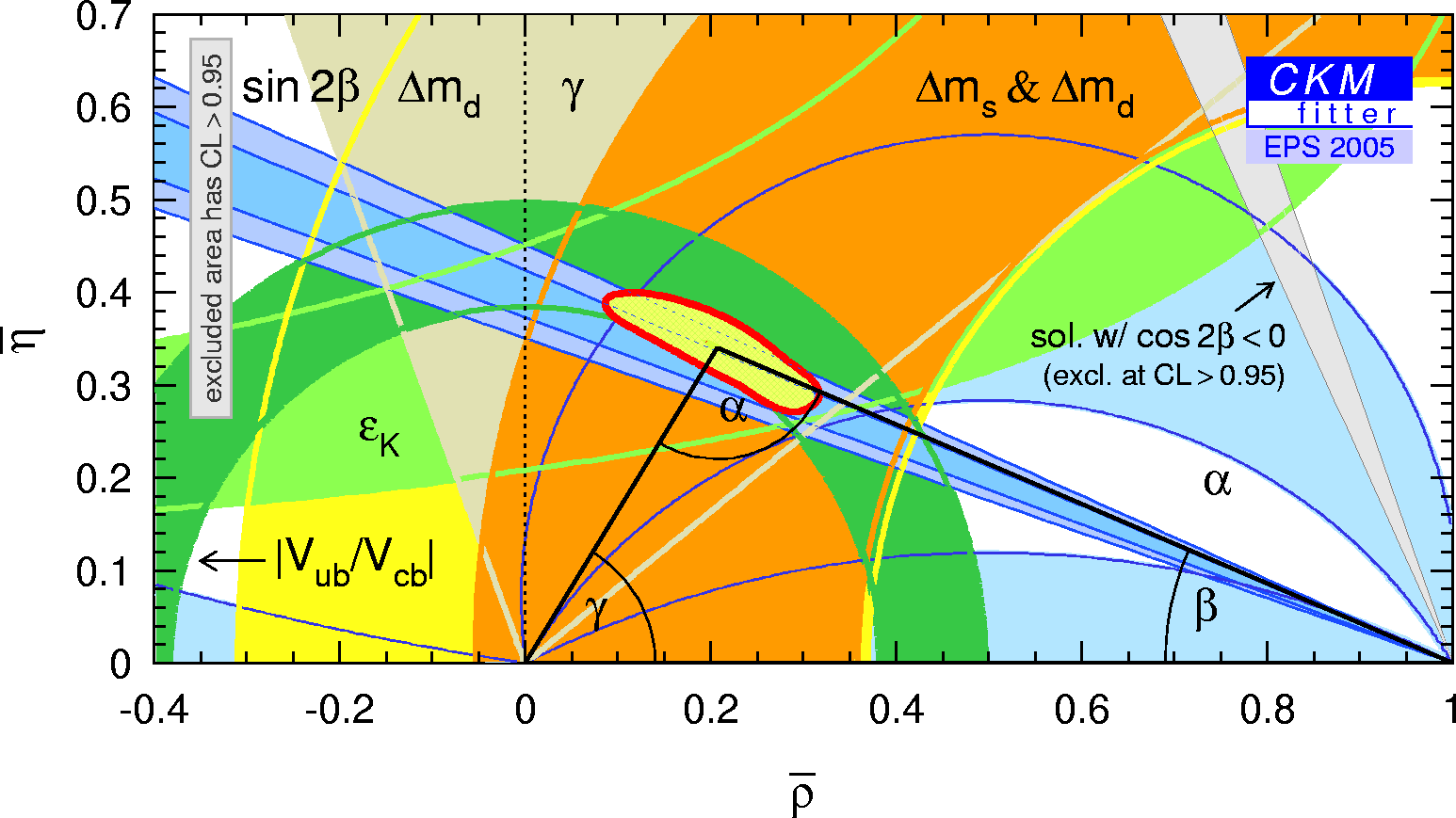eps gif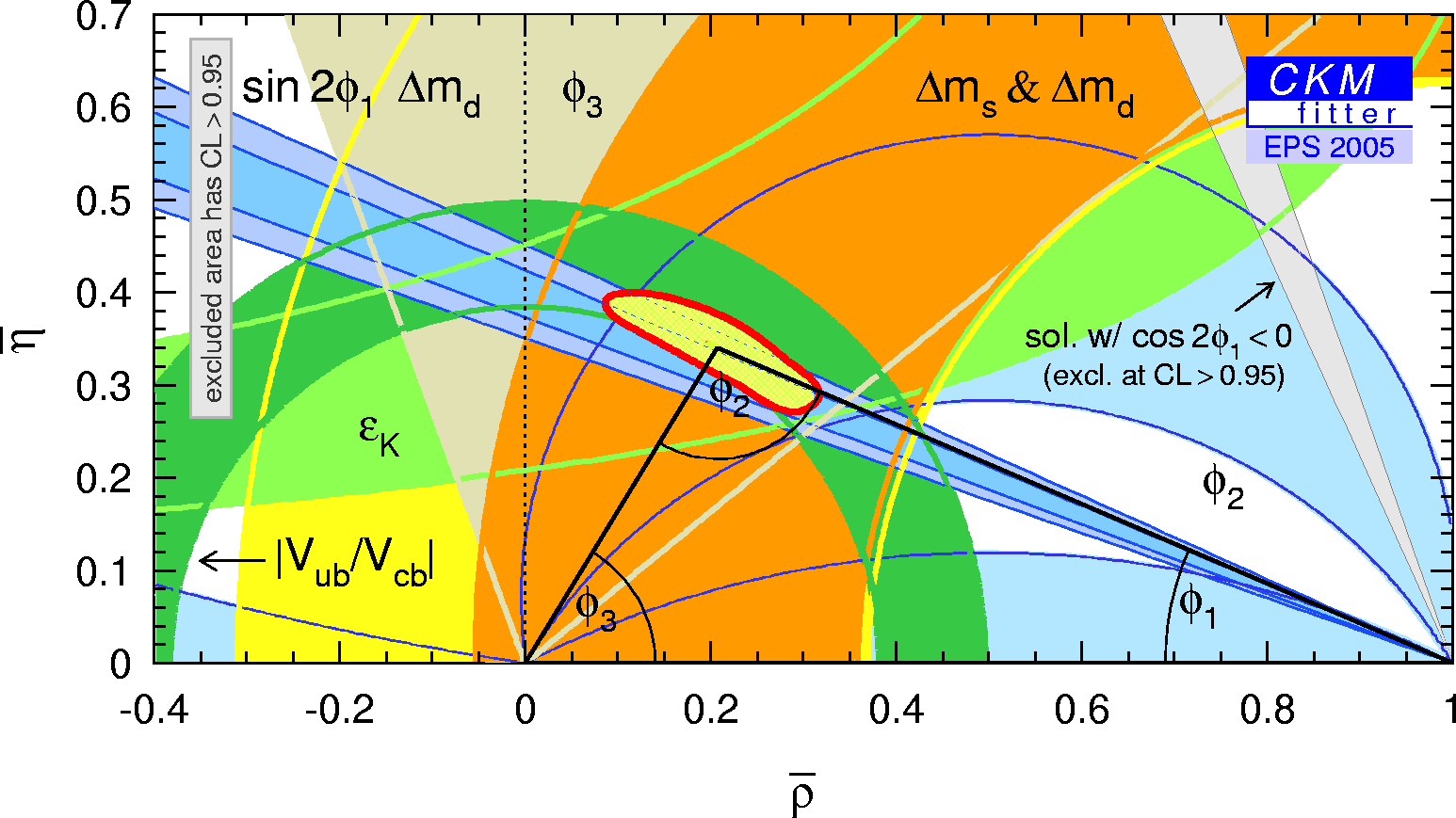eps gif Zoomed constraints in the (ρ-bar,η-bar) plane not including the angle measurements in the global fit.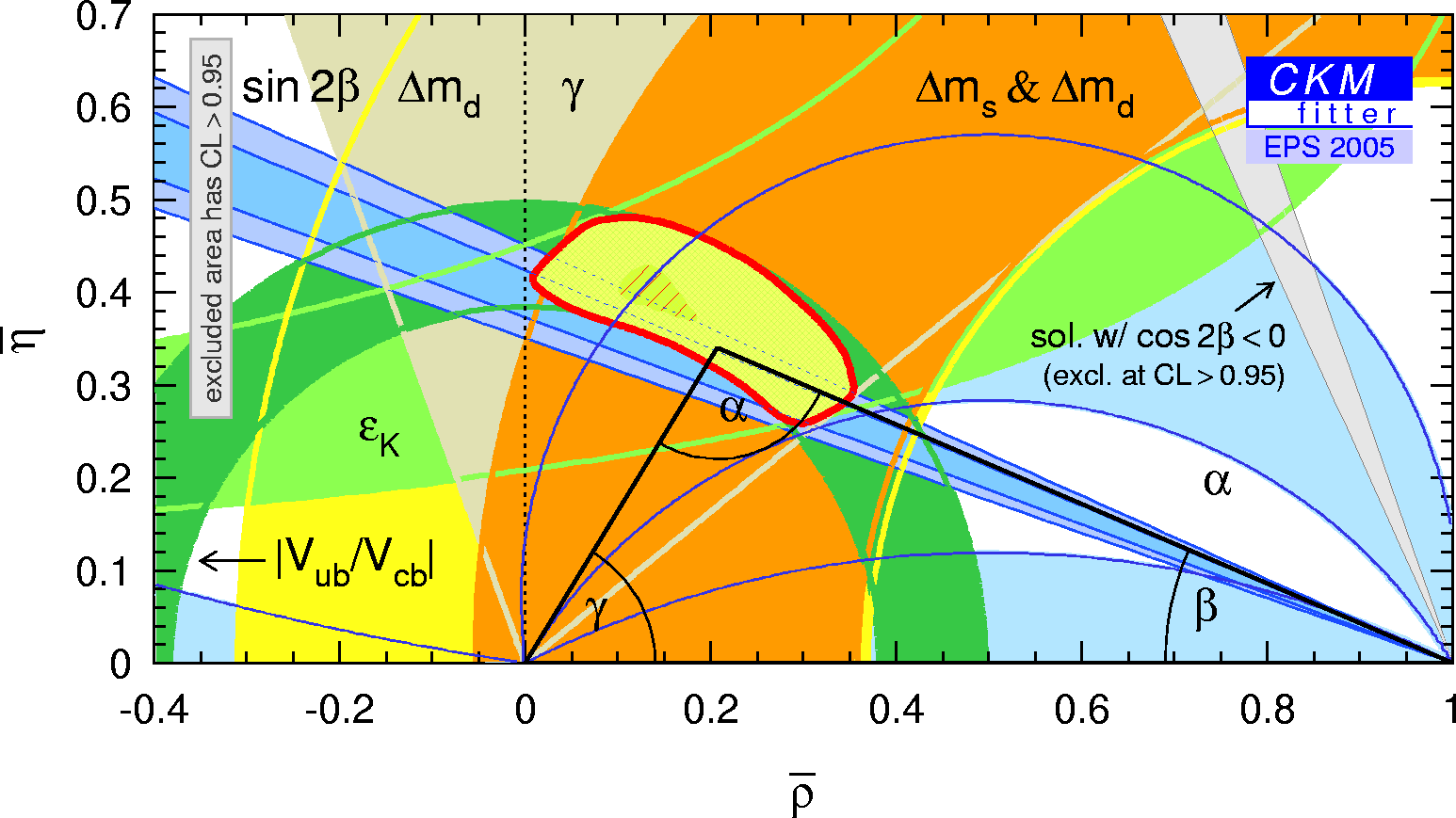eps gif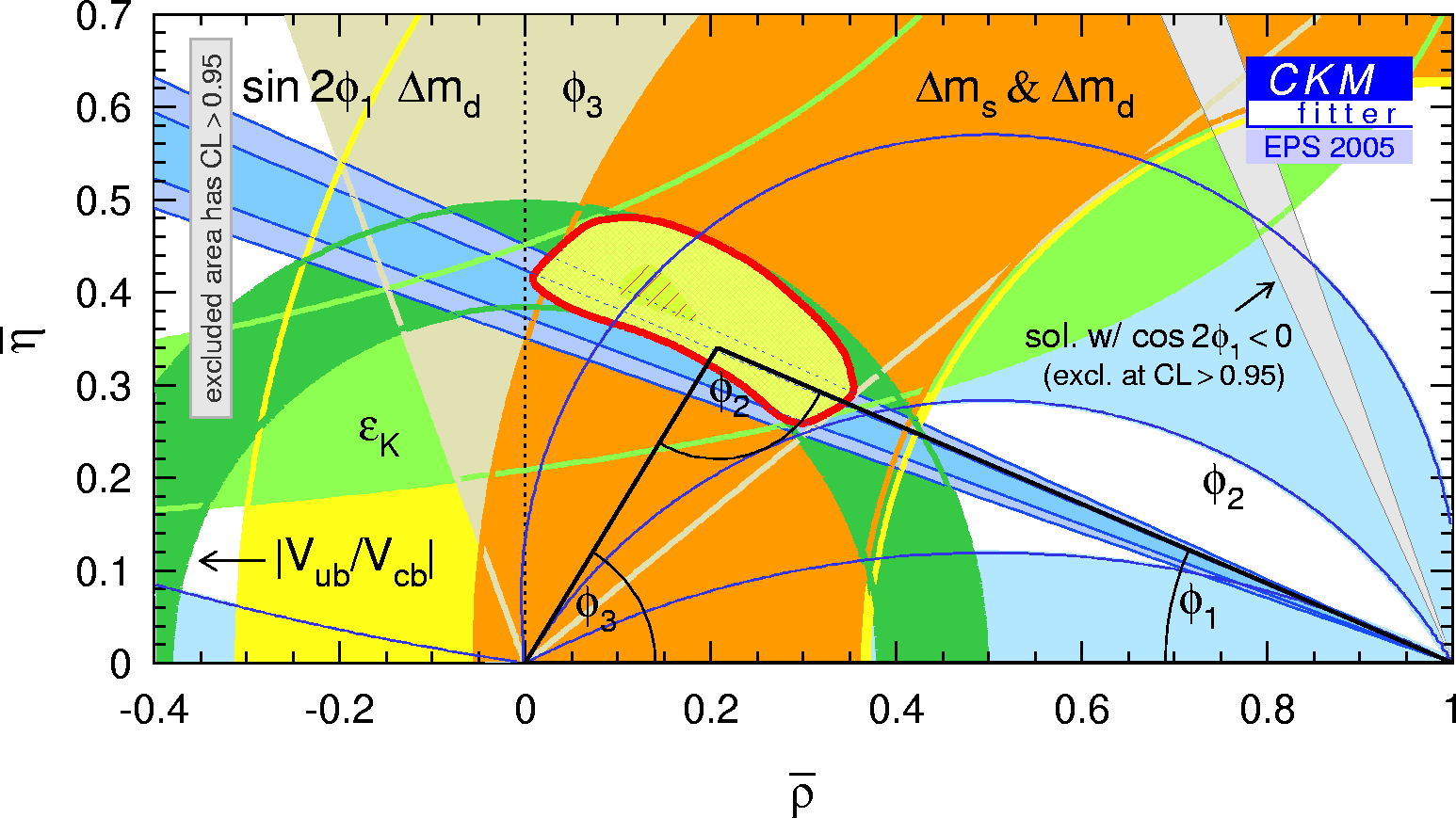eps gif Constraints in the (ρ-bar,η-bar) plane including only the angle measurements in the global fit.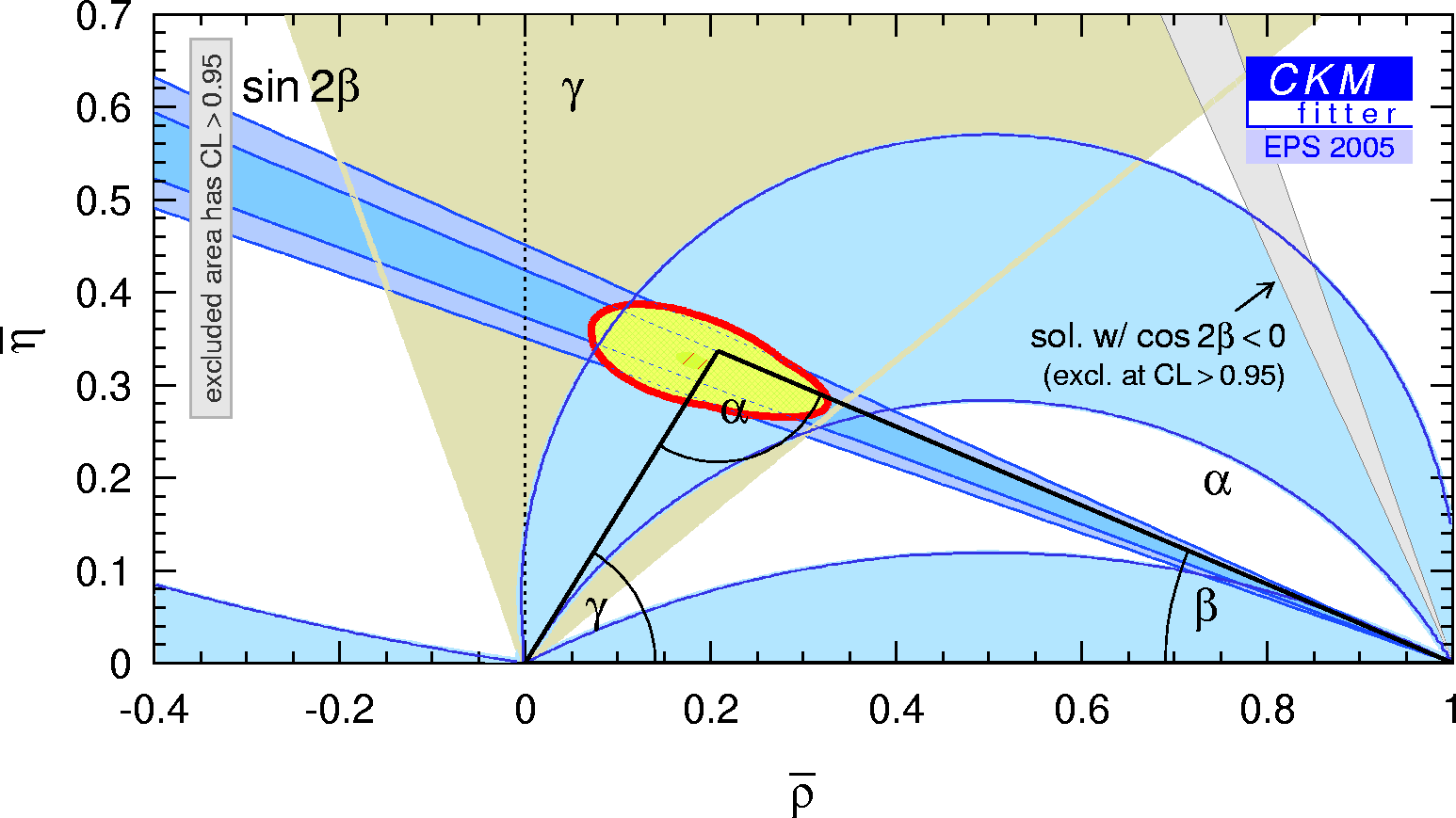eps gif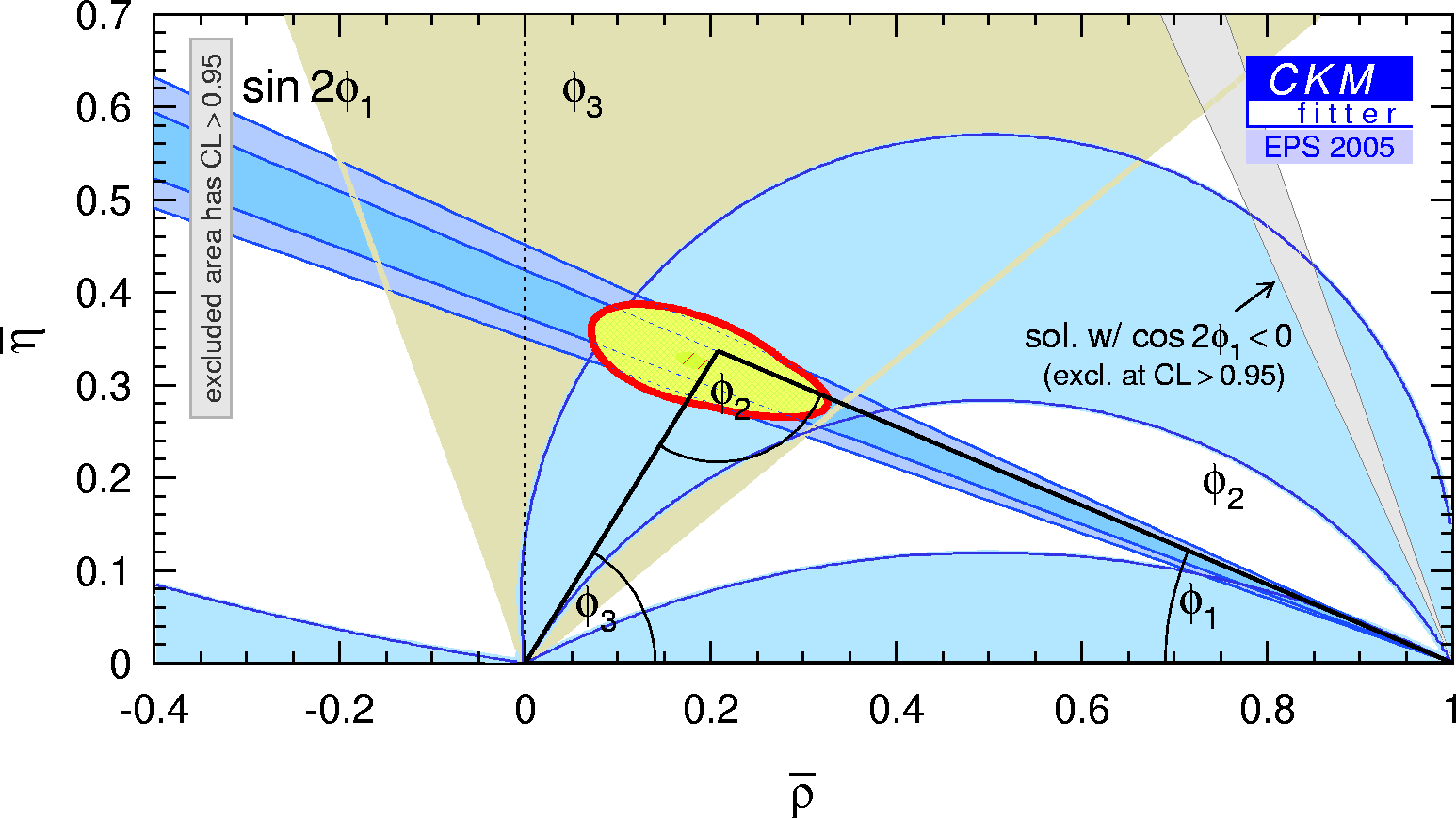eps gif

 Constraint on α/Φ2 from B→ππ.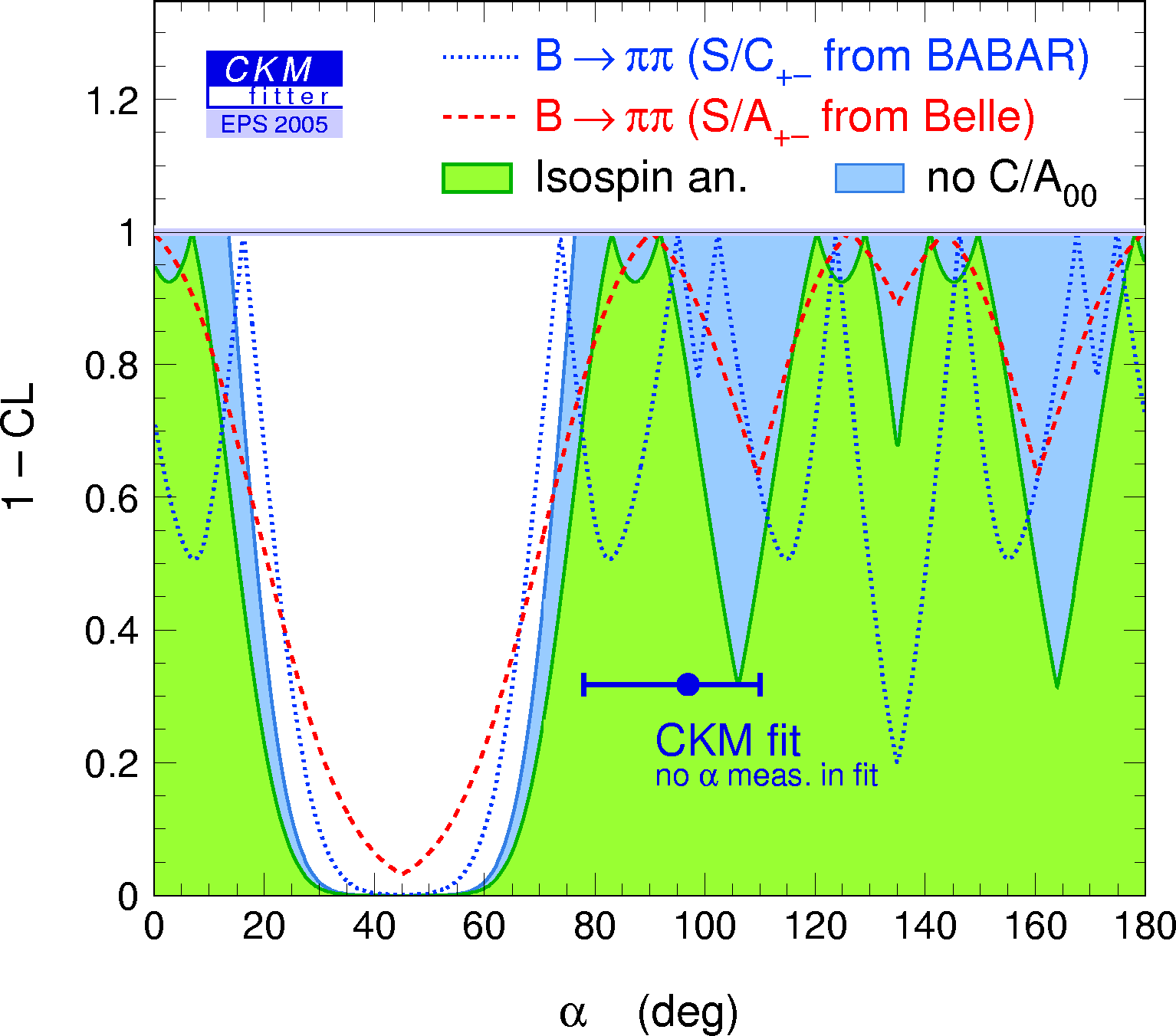eps gif Constraint on α/Φ2 from B→ρρ.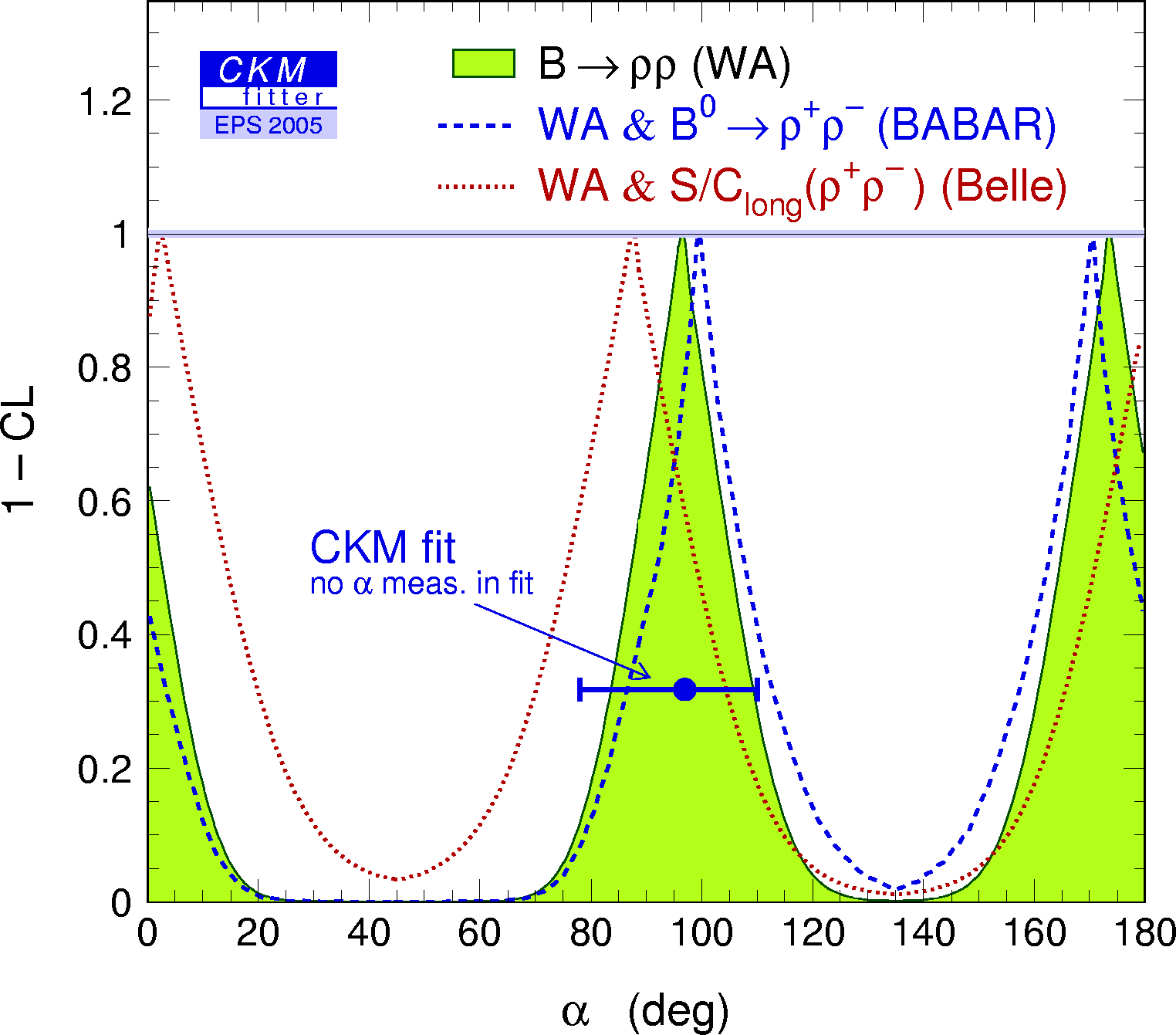eps gif Constraints on α/Φ2 from B→ππ, ρπ, ρρ, compared to the prediction from the CKM fit (not including these measurements). α[combined] = 98.6 +12.6/–8.1 deg.eps gif Constraint in the (ρ-bar,η-bar) plane from B→ππ, ρπ, ρρ, compared with the global CKM fit (not including these α-related measurements).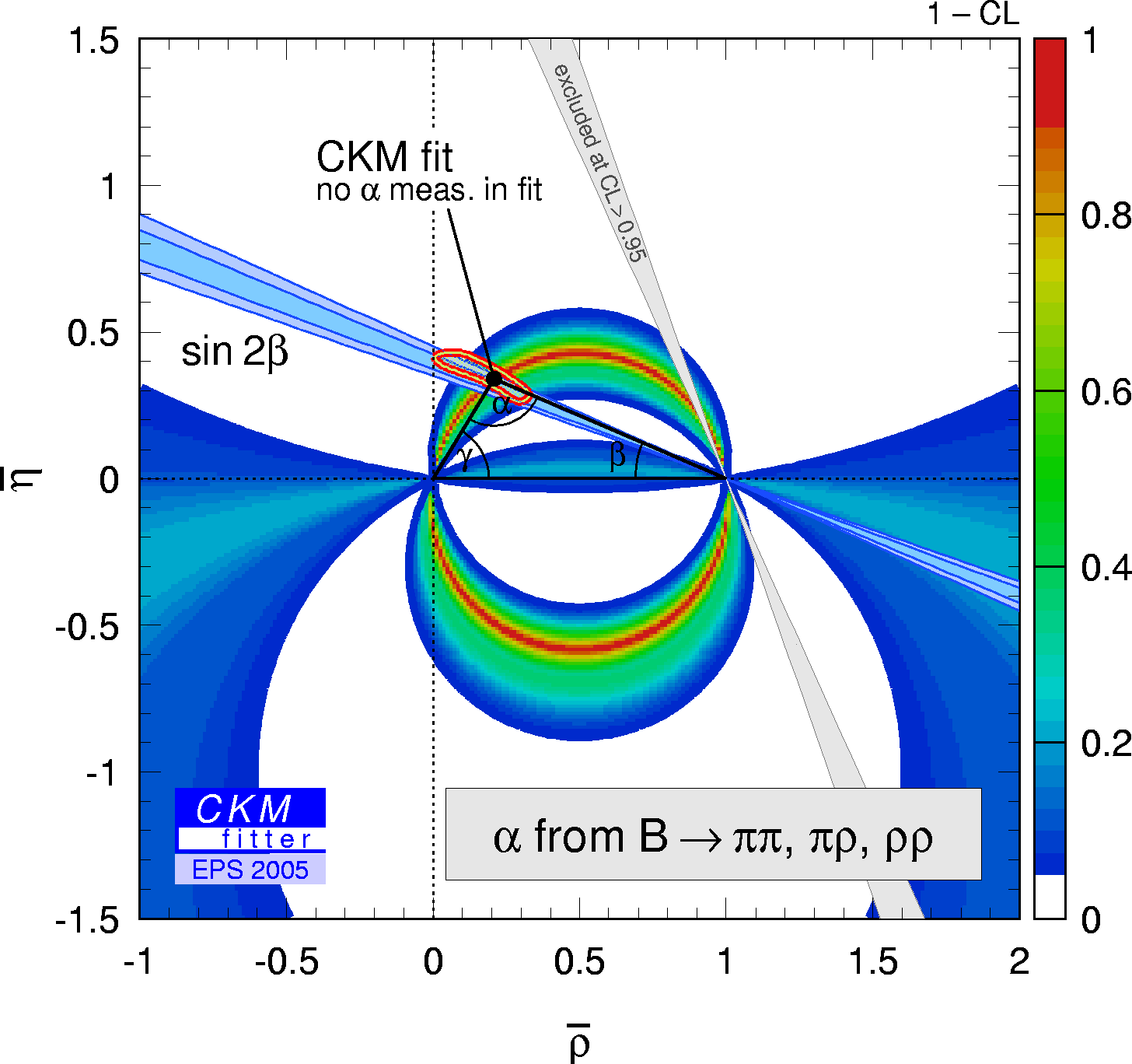eps gif The numerical results for these quantities are given in thesummary paper.

 Constraints on γ/Φ3 from world average D(*)K decays (GLW+ADS) and Dalitz analyses compared to the prediction from the global CKM fit (not including these measurements). γ[combined] = 63 +15/–12 deg.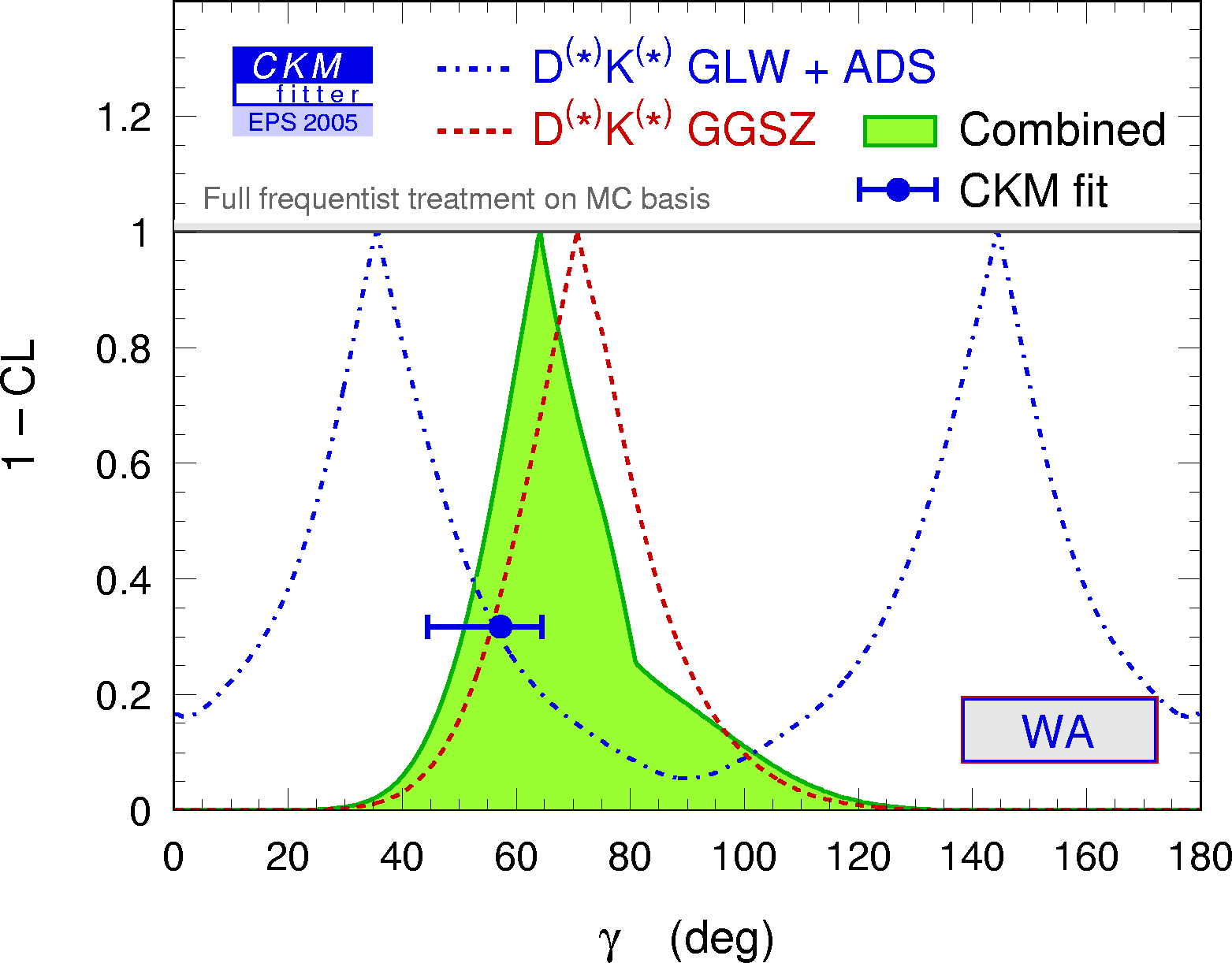eps gif Constraints on γ/Φ3 from world average D(*)K decays (GLW+ADS) and Dalitz analyses from BABAR compared to the prediction from the global CKM fit (not including these measurements).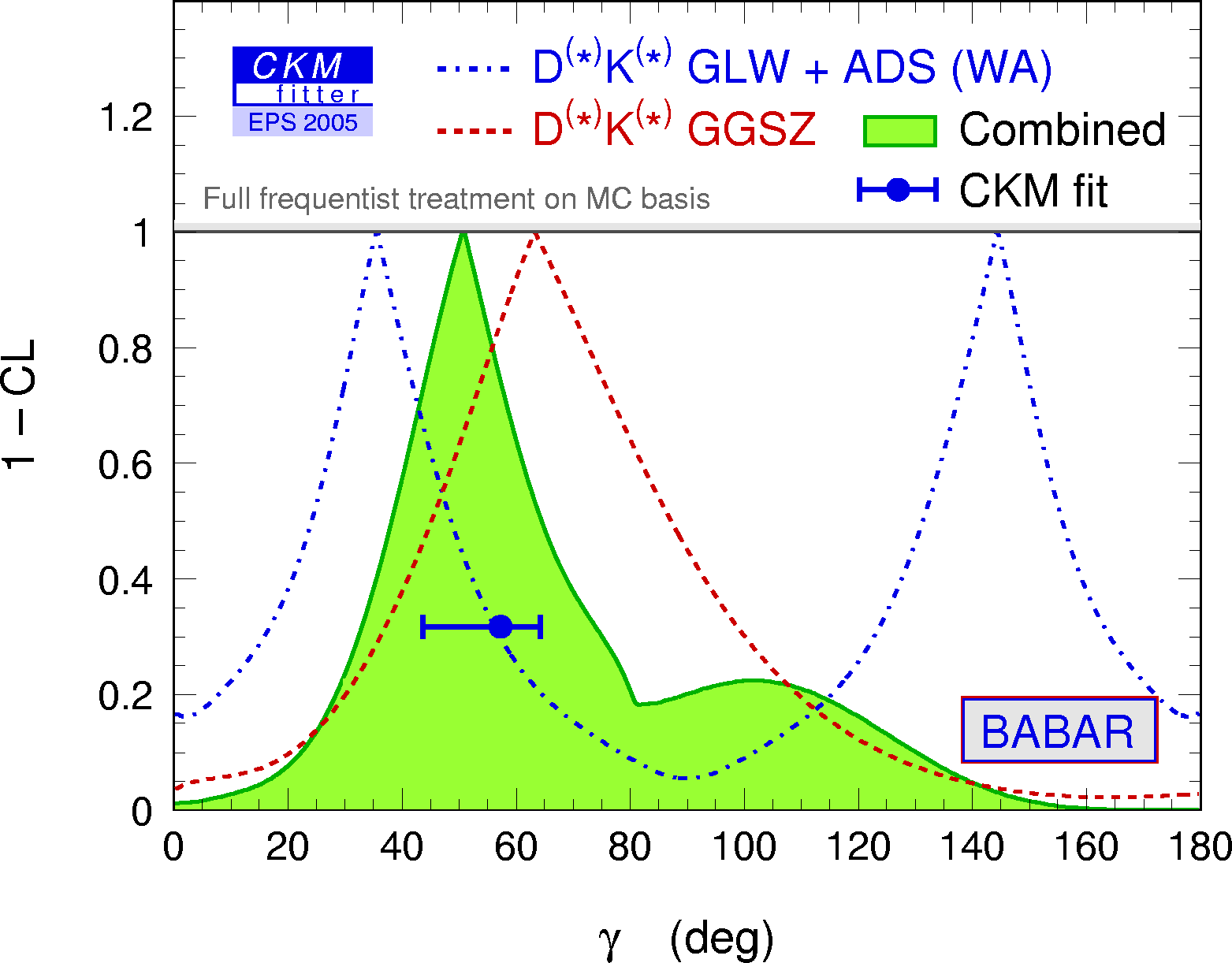eps gif α,β,γ convention: φ1,φ2,φ3 convention: Constraints in the (ρ-bar,η-bar) plane on γ/Φ3 from world average D(*)K decays (GLW+ADS) and Dalitz analyses compared to the prediction from the global CKM fit (not including these measurements).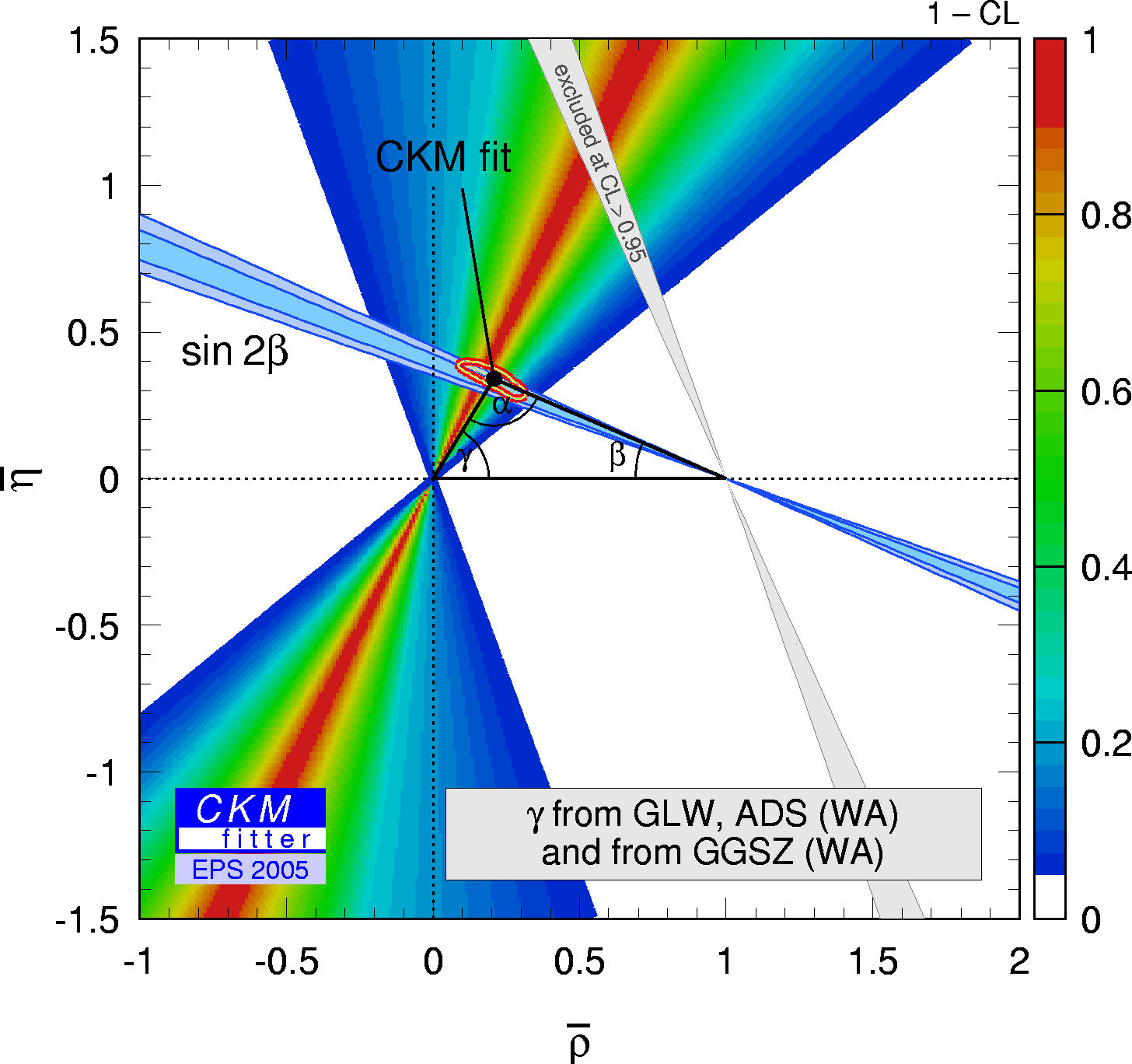eps gif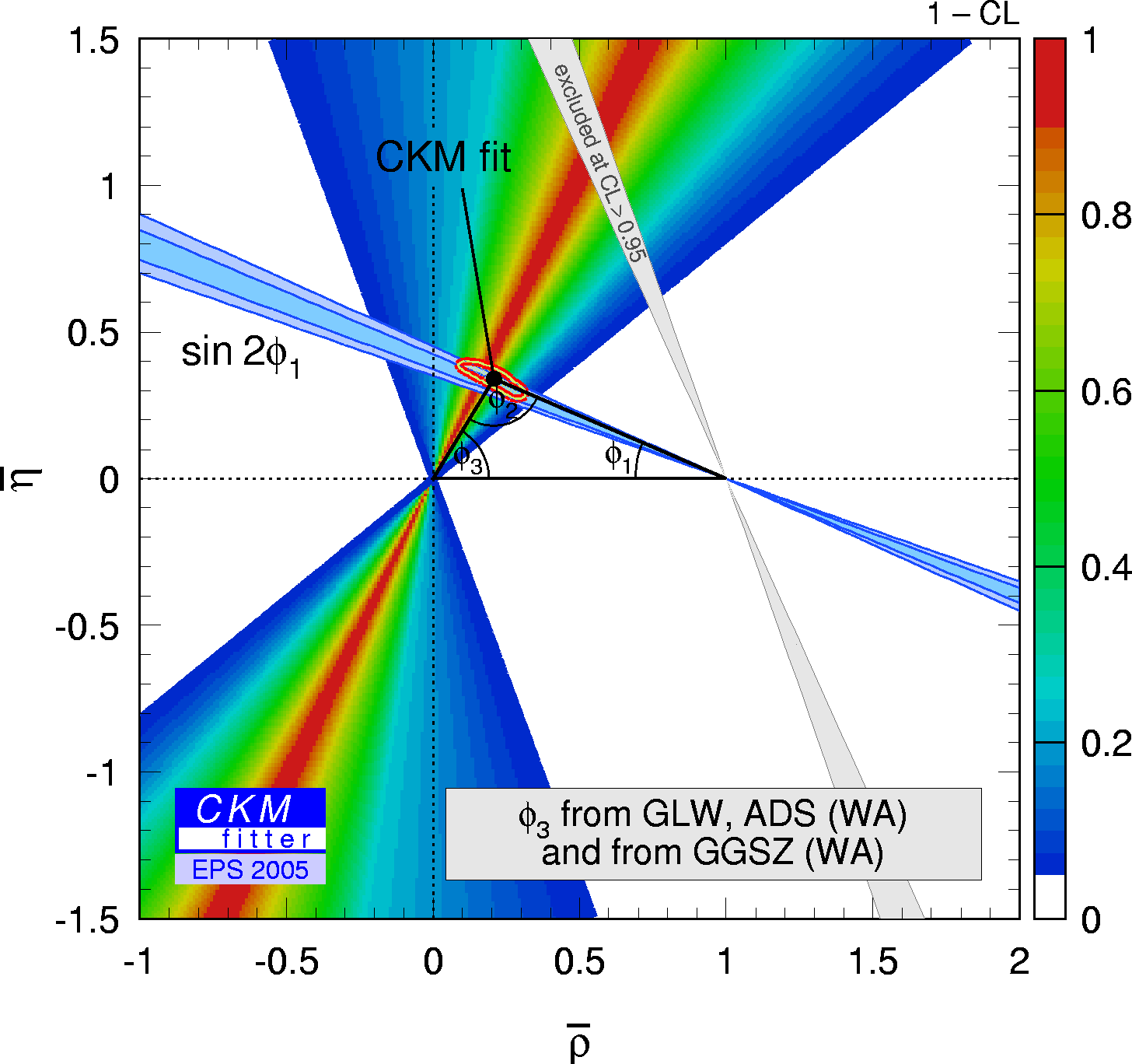eps gif Constraints in the (ρ-bar,η-bar) plane on γ/Φ3 from world average D(*)K decays (GLW+ADS) and Dalitz analyses from BABAR compared to the prediction from the global CKM fit (not including these measurements).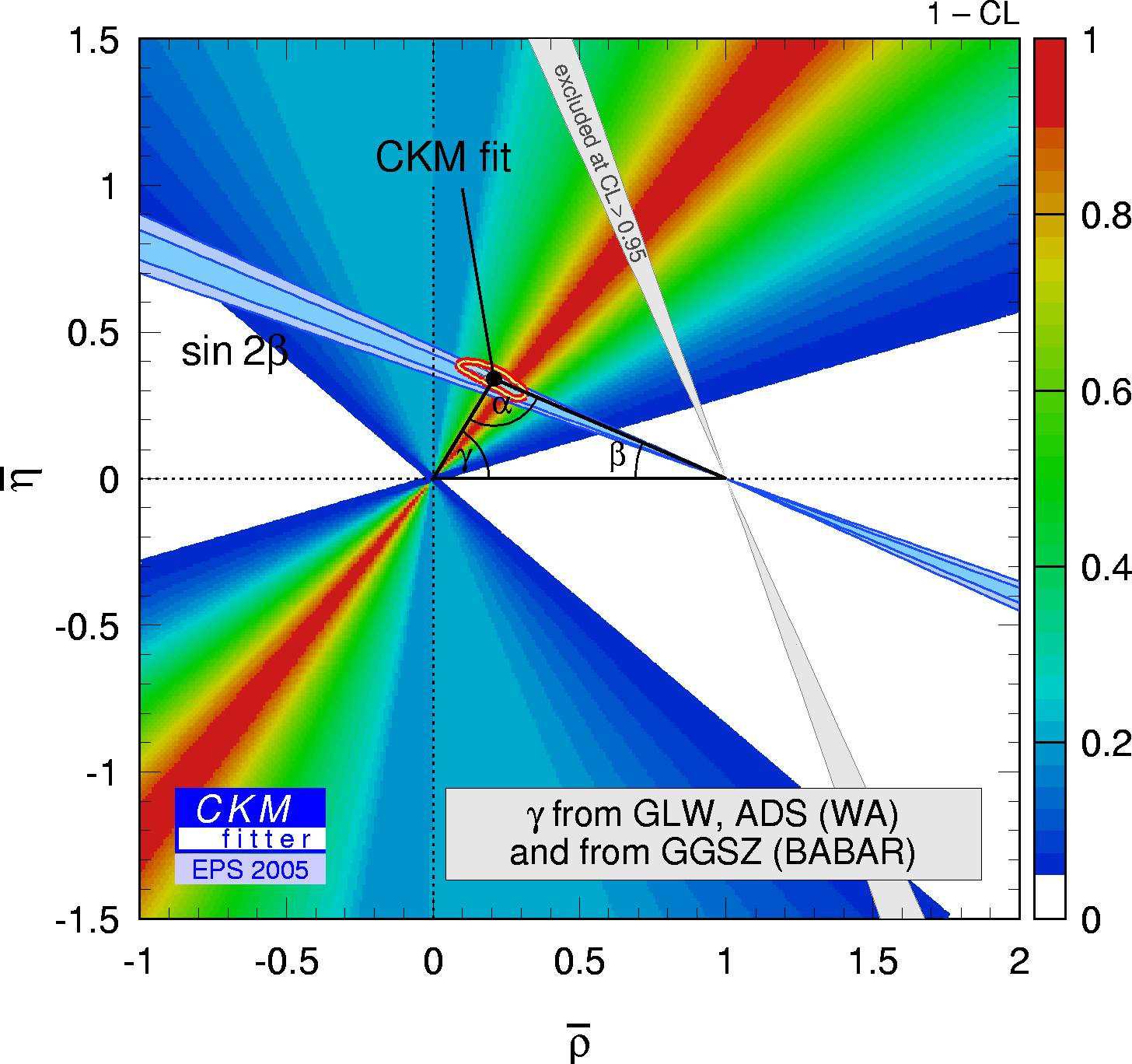eps gif The numerical results for these quantities are given in thesummary paper.

 Constraints on the angle βs =arg(-VtsVtb*/VcsVcb*) (upper plot) and sin(2βs) (lower plot) from the global CKM fit (no direct measurement from the time-dependent CP asymmetry in B0→J/ψφ decays is available yet).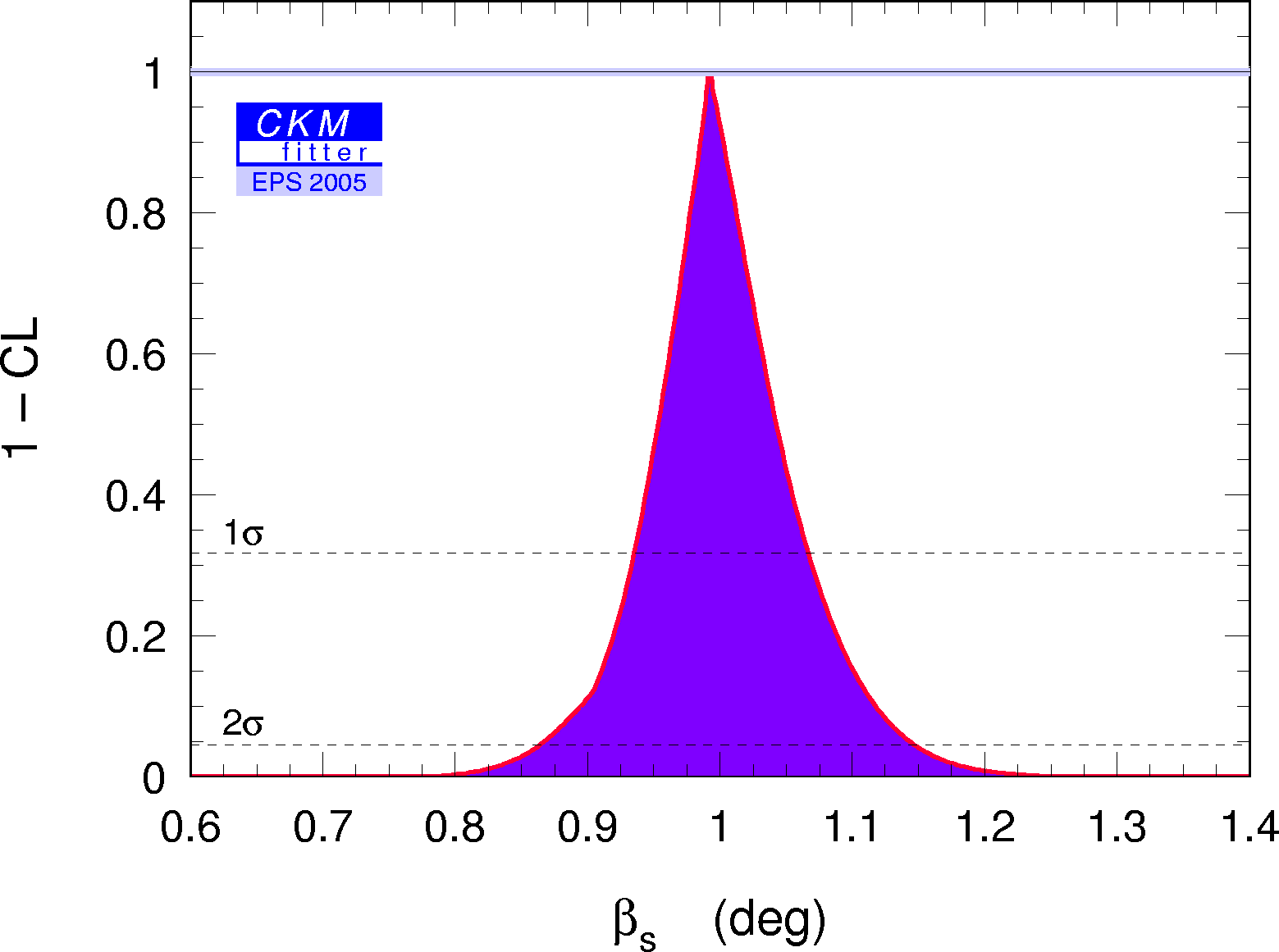eps gif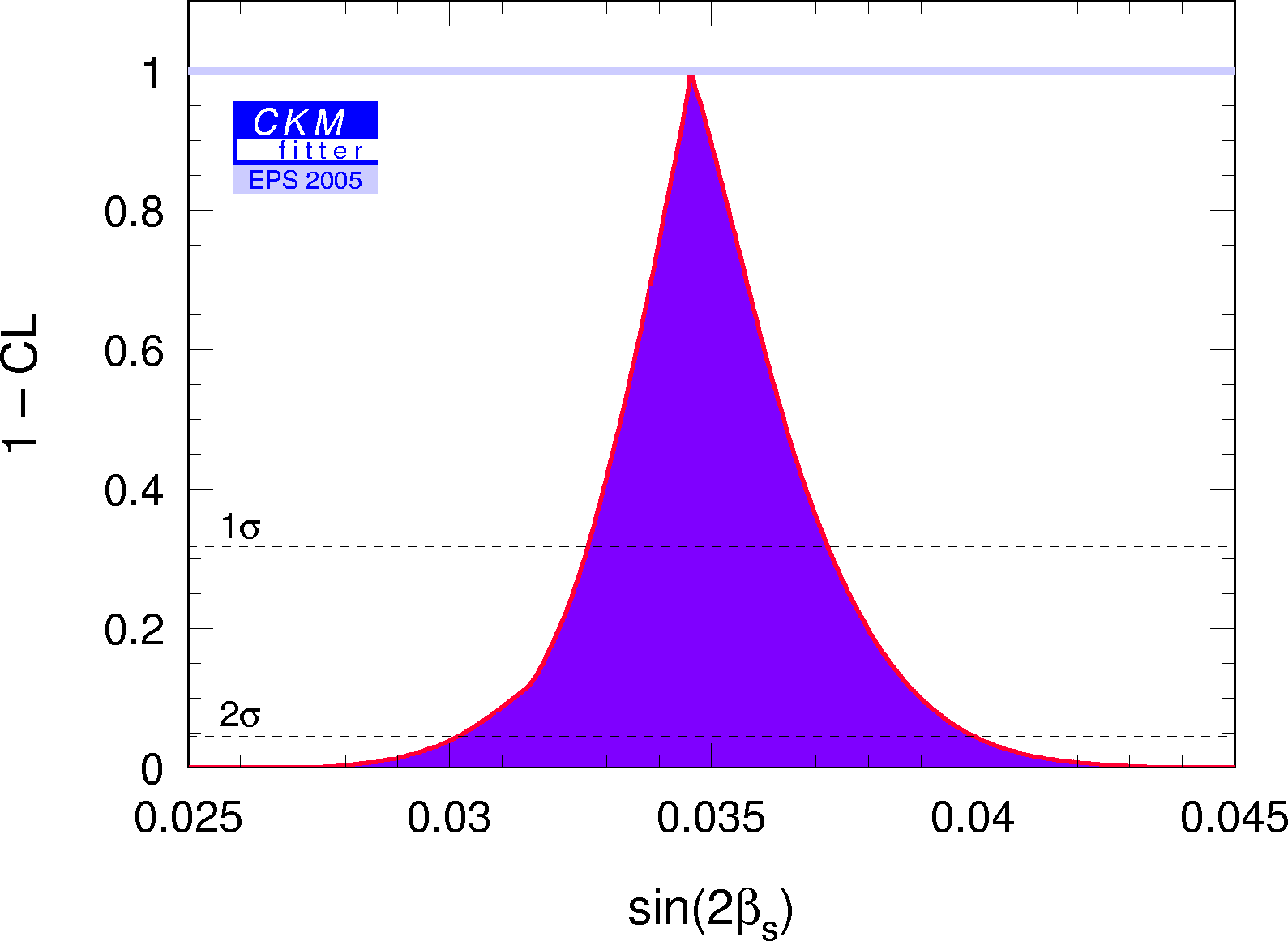eps gif The numerical results for these quantities are given in thesummary paper.

 Constraint in the (ρ-bar,η-bar) plane from the simultaneous use of the limit on the B+→τ+ν branching fraction and Δmd.eps gif Constraint in the (ρ-bar,η-bar) plane from the simultaneous use of the limit on the B+→τ+ν branching fraction and Δmd. Also shown is the constraint one would obtain by a precise measurement of BR(B+->tau+nu). It illustrates the gain in accuracy obtained from the simultaneous use of the two constraints ( B+→τ+ν and Δmd), where the uncertainty from fB is reduced (it cancels in the ratio).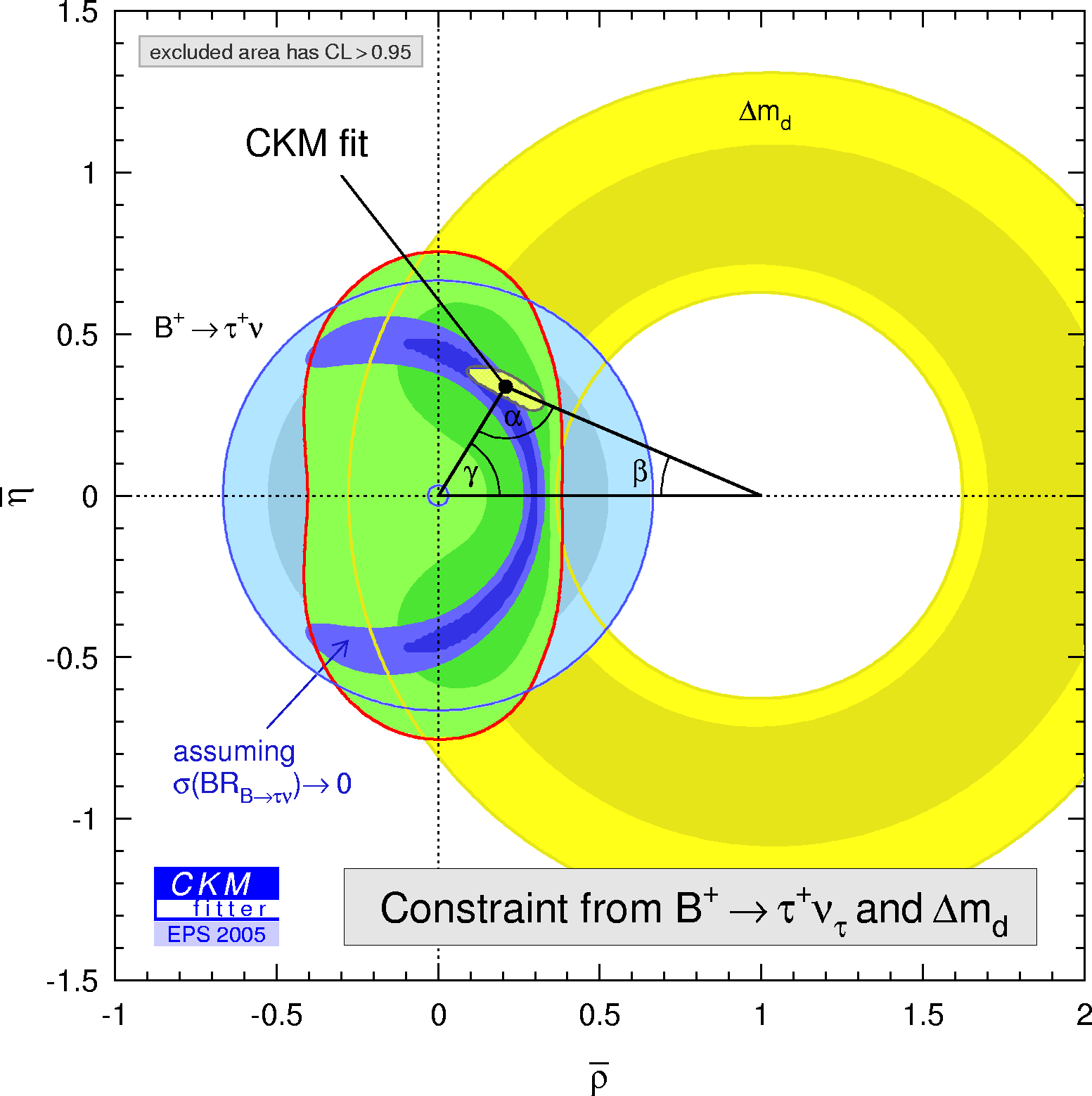eps gif

 Constraint in the (ρ-bar,η-bar) plane from the branching fraction (B0→ρ0 γ / B0→K*0 γ). Average BABAR and Belle.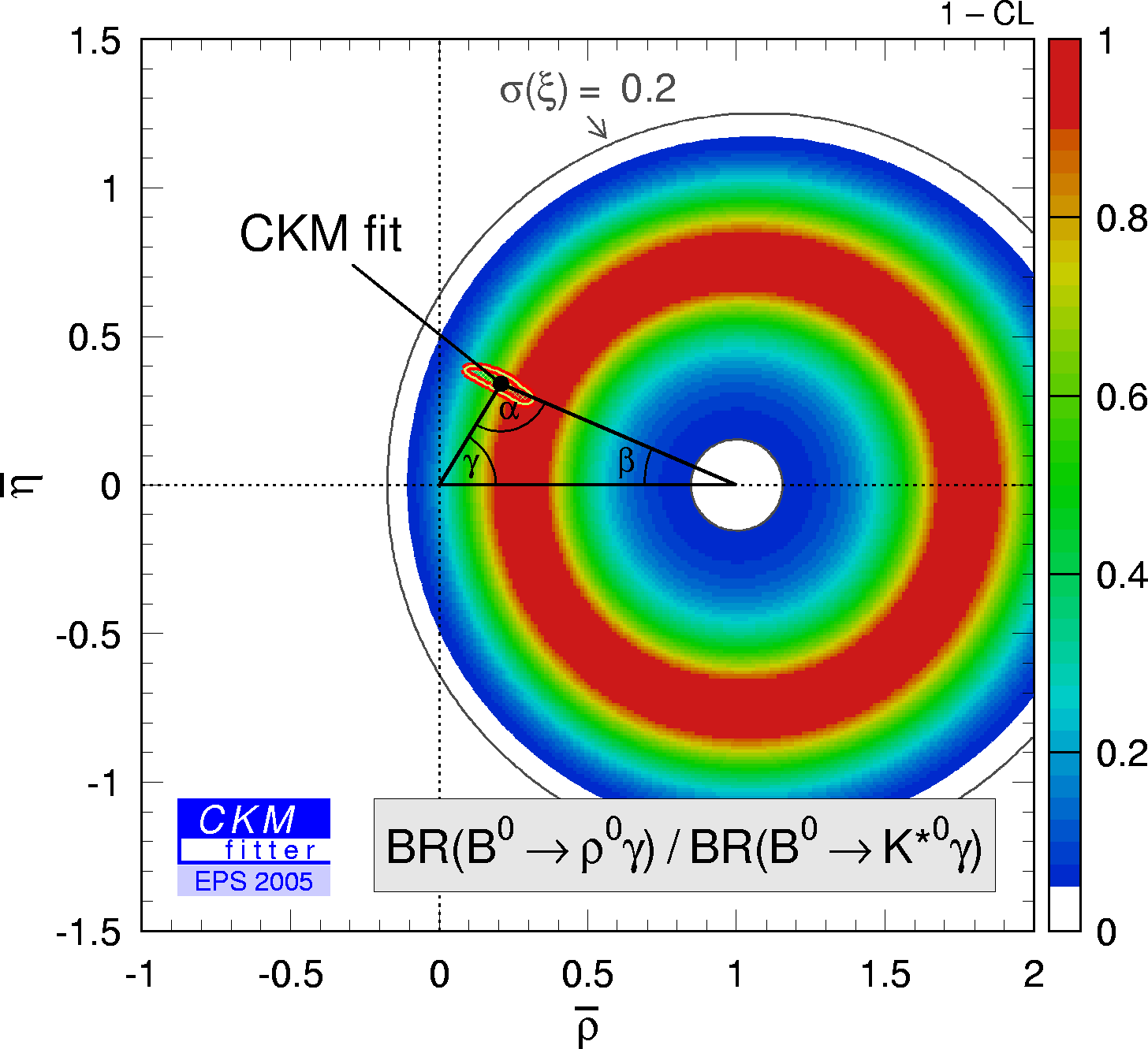eps gif Constraint in the (ρ-bar,η-bar) plane (not including the angle measurements in the global fit) from the branching fraction (B0→ρ0 γ / B0→K*0 γ). Average BABAR and Belle.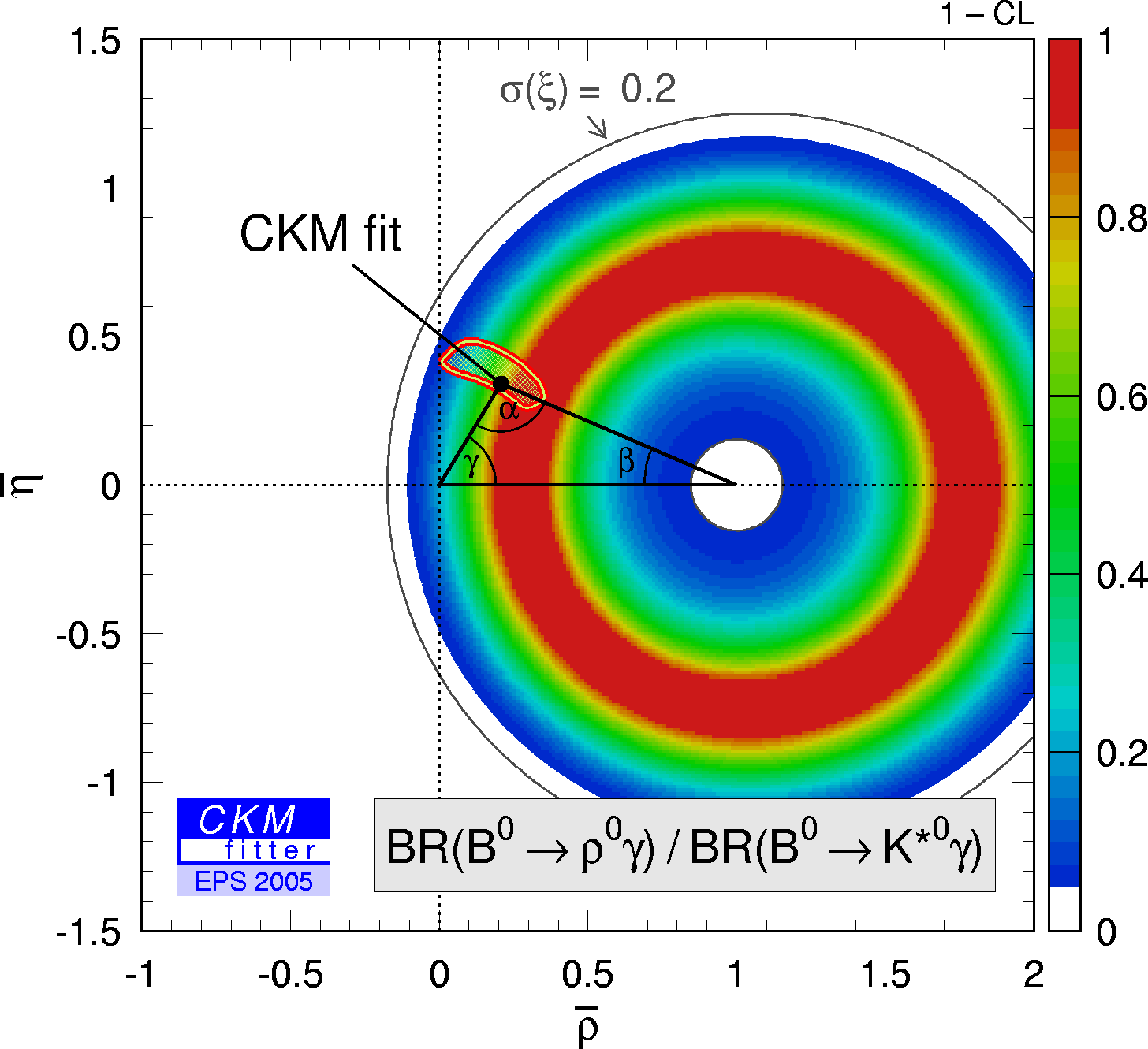eps gif Constraint in the (ρ-bar,η-bar) plane from the branching fraction (B0→ρ0 γ / B0→K*0 γ). BABAR only.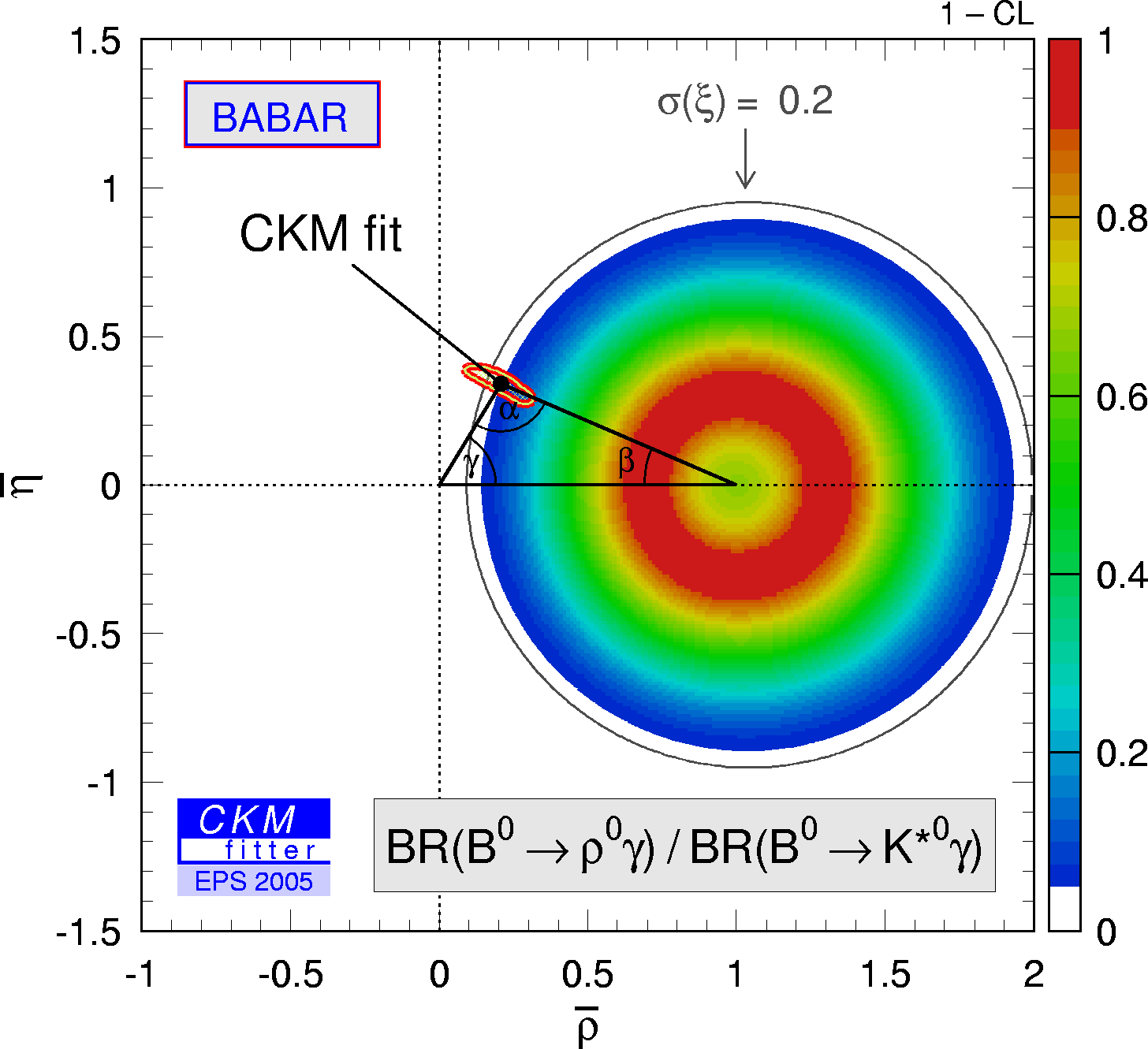eps gif Constraint in the (ρ-bar,η-bar) plane from the ratio of the branching fractions (B→ργ / B→K* γ) where the average of neutral and charged B decays (and average of BABAR and Belle) has been used.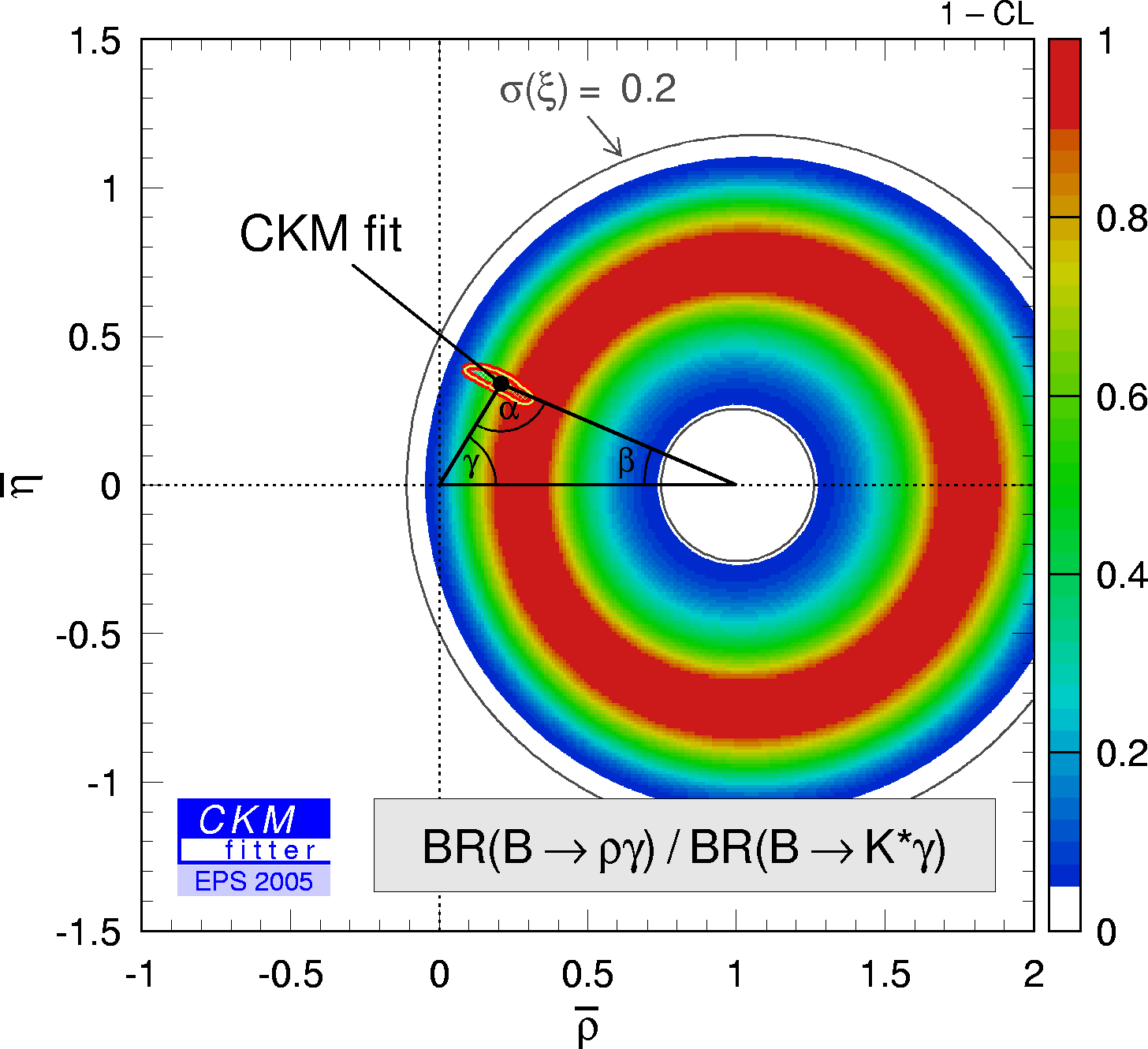eps gif Constraint in the (ρ-bar,η-bar) plane (not including the angle measurements in the global fit) from the ratio of the branching fractions (B→ργ / B→K* γ) where the average of neutral and charged B decays (and average of BABAR and Belle) has been used.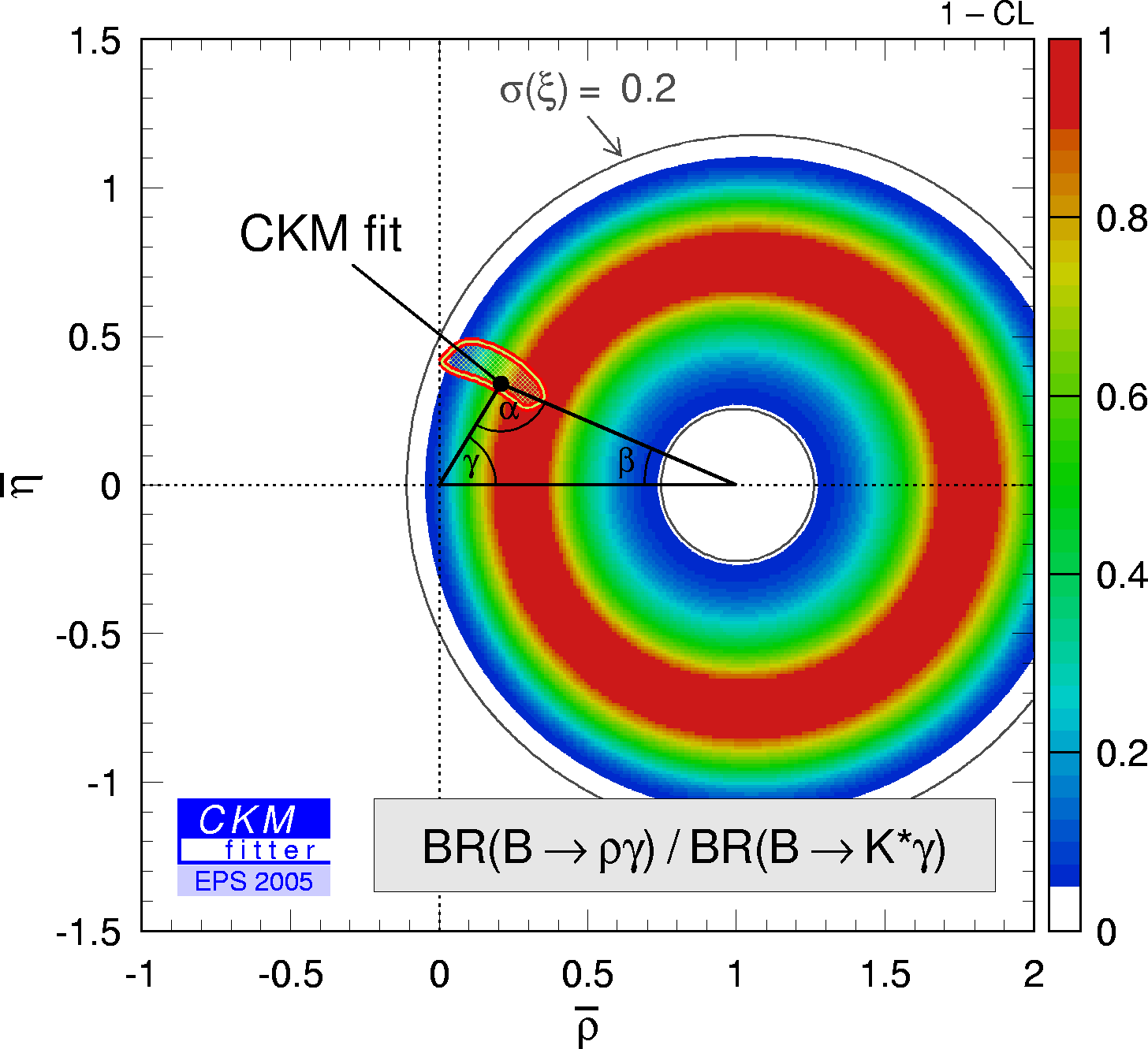eps gif

 Constraints on |sin(2β+γ)| from the measurement of time-dependent CP asymmetries in D(*) π (ρ). Moriond05 HFAG average is used as input. The extraction of the UT angles relies on SU(3) symmetry for the estimates of the suppressed-to-leading amplitude ratios. We use r = 0.019 ± 0.004 and r* = 0.015 + 0.004 / – 0.006 , and apply an additional theoretical uncertainty in form of a 30% error range to these.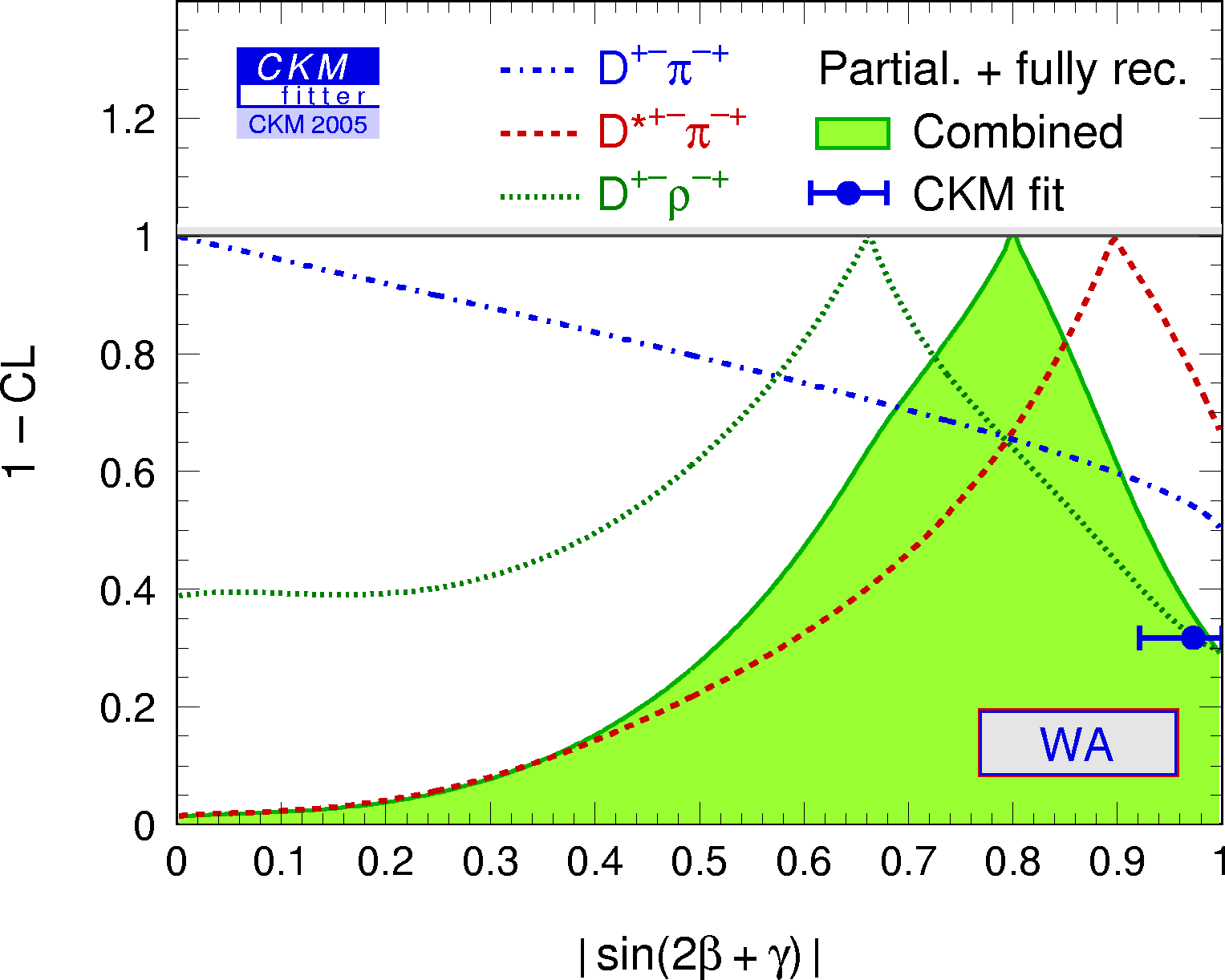eps gif Translation of this result into γ (using sin(2β) as additional input and choosing among the four solutions to the SM one). γ[GLW+ADS+GGSZ+|sin(2β+γ)|] = 70 +12 / – 14°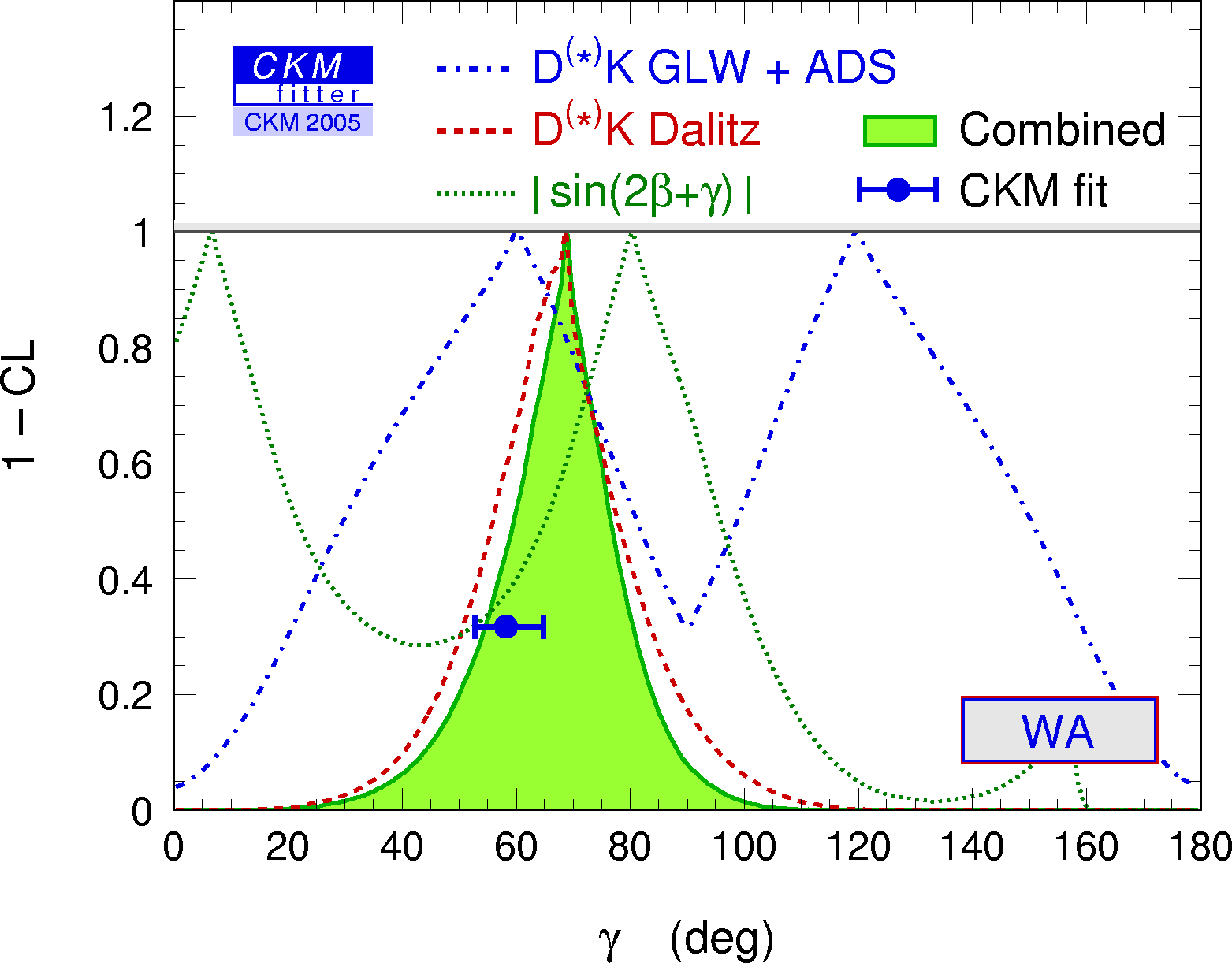eps gif Constraints from |sin(2β+γ)| in the (ρ-bar,η-bar) plane.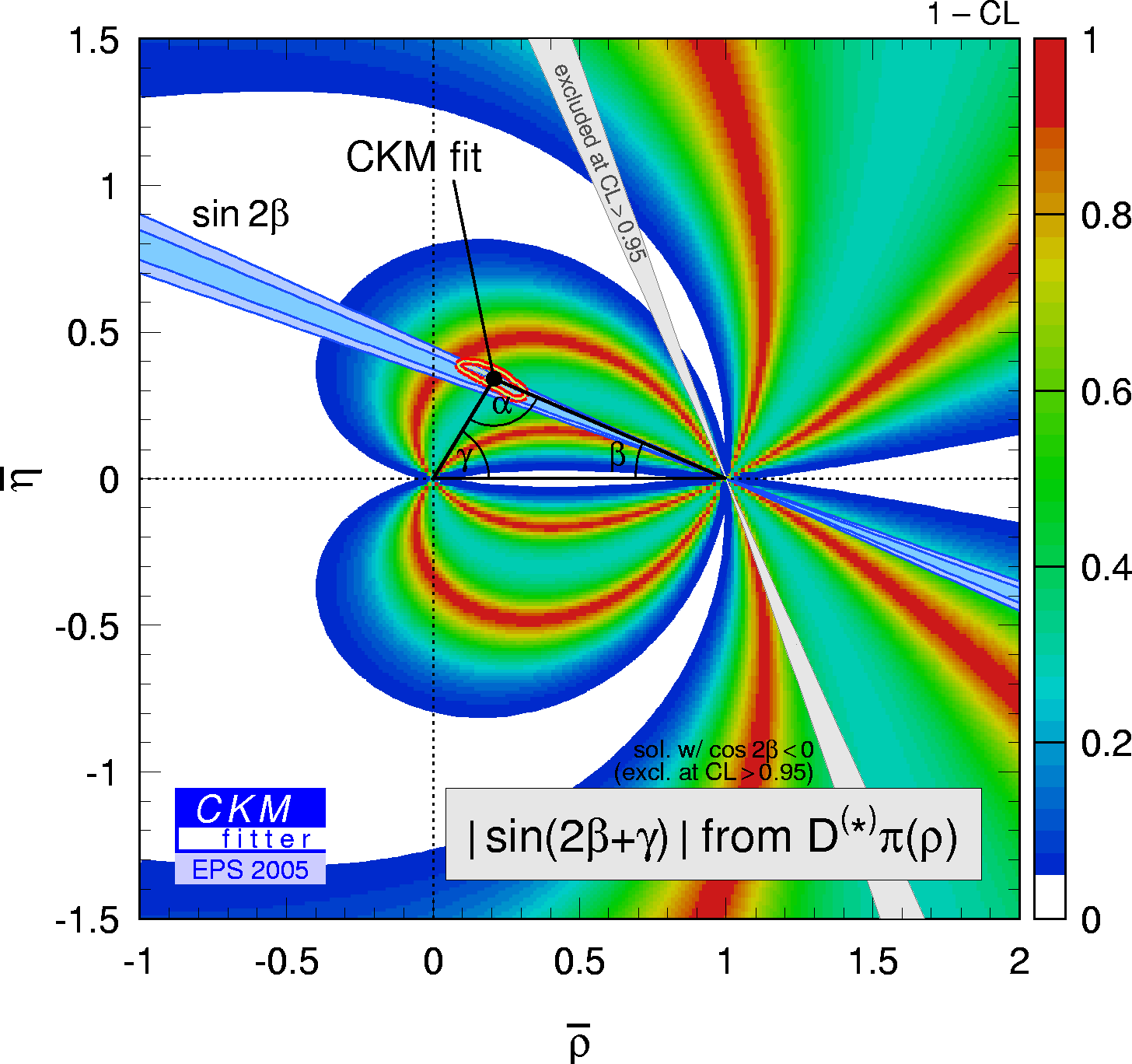eps gif

 Constraints from CP conserving quantities (|Vub / Vcb|, Δmd, (Δmd and Δms) and B+→τ+ ν) in the (ρ-bar,η-bar) plane.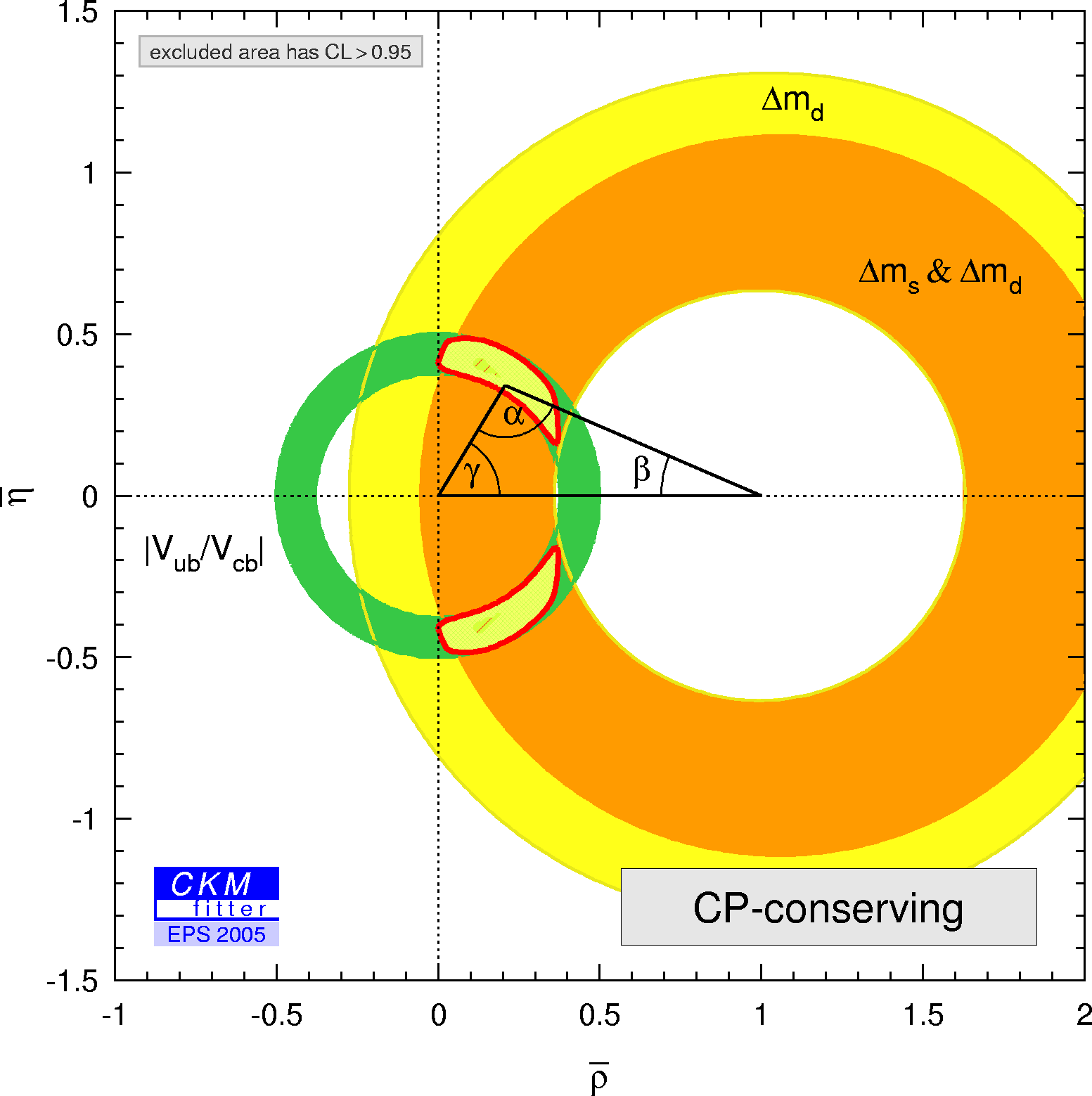eps gif Constraints from CP violating quantities (sin(2β), α, γ and εk) in the (ρ-bar,η-bar) plane.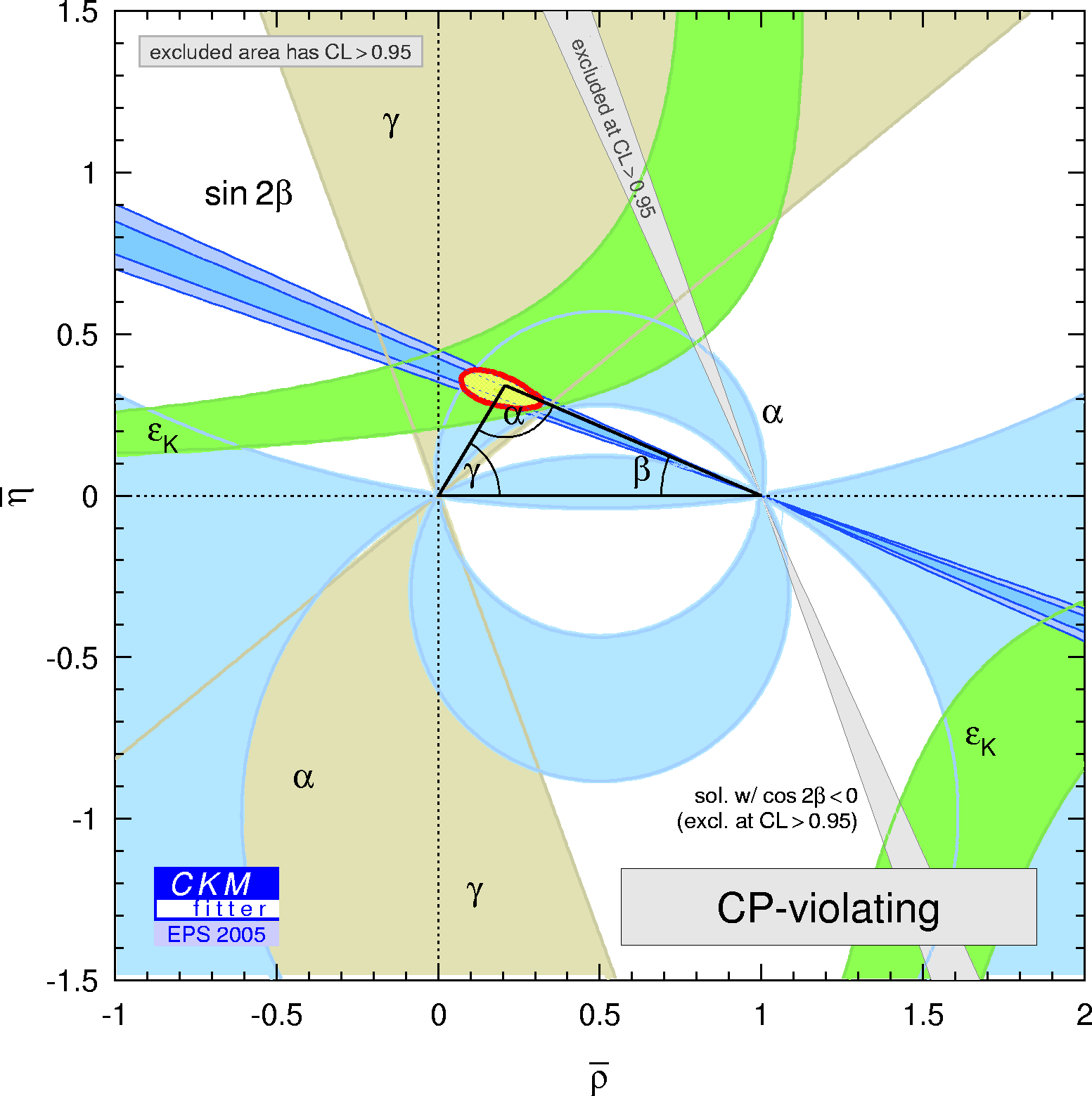eps gif Constraints from |Vub / Vcb| and s to d transition in the (ρ-bar,η-bar) plane.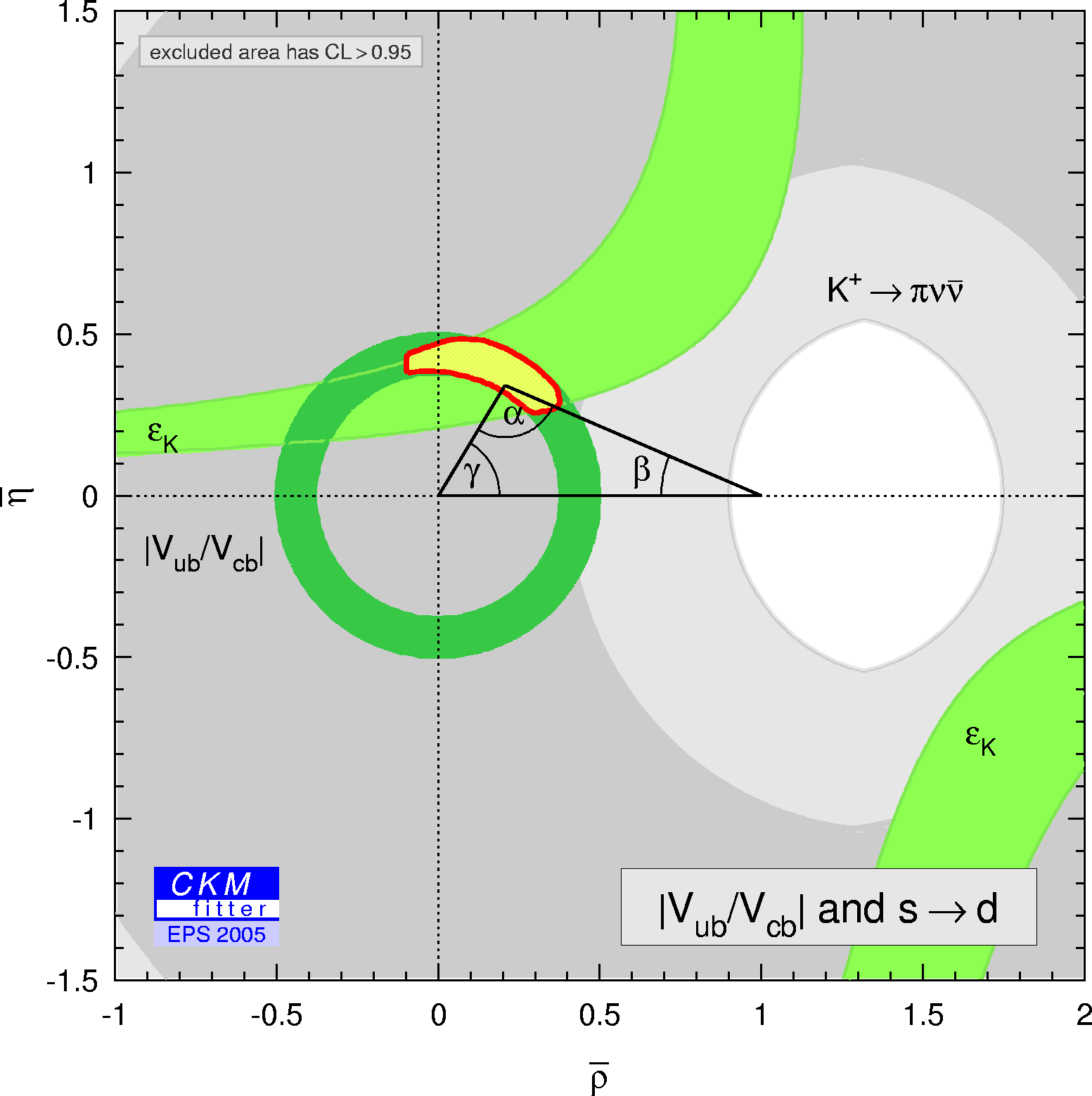eps gif Constraints from |Vub / Vcb| and b to d transition in the (ρ-bar,η-bar) plane.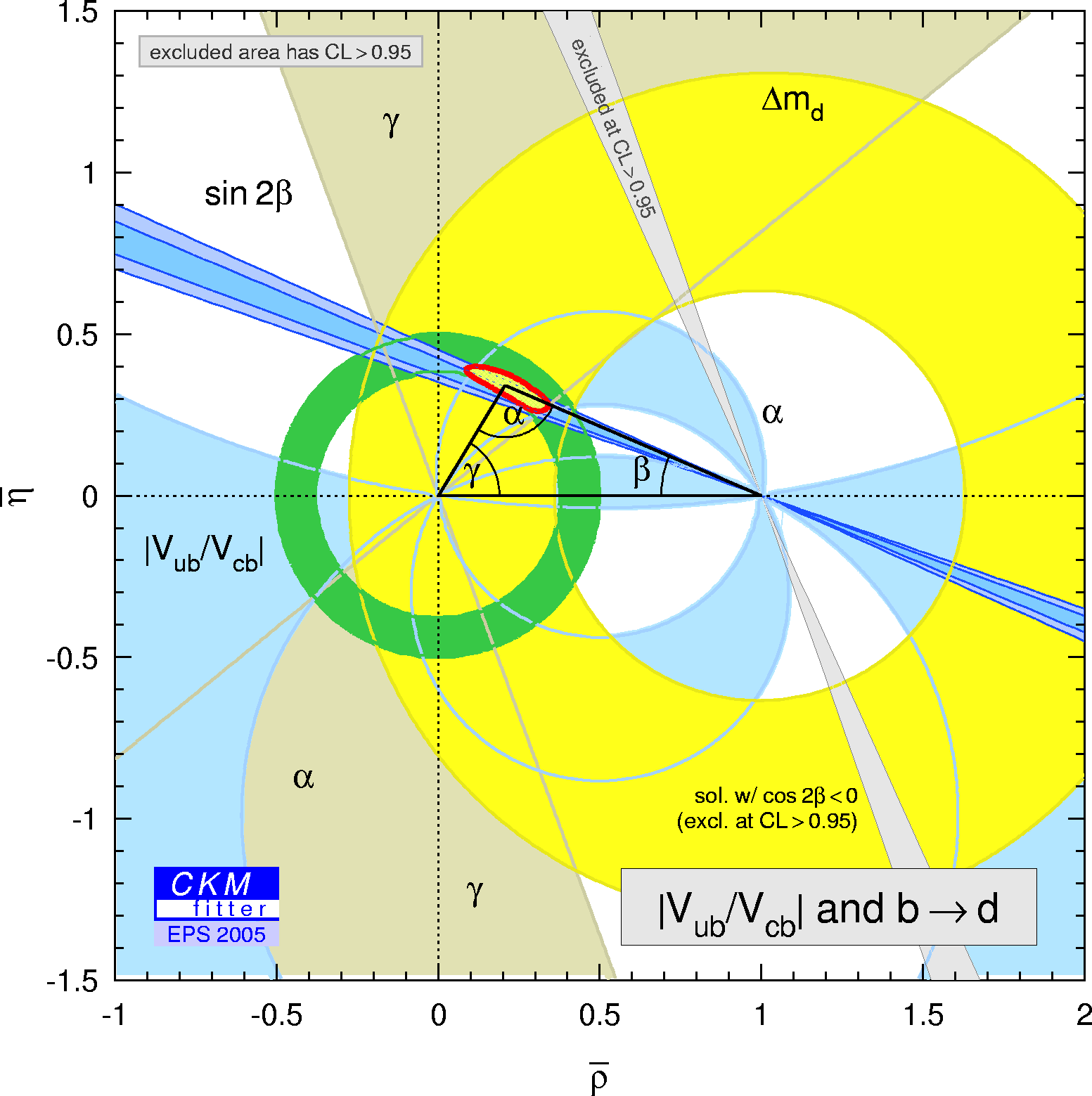eps gif Constraints from |Vub / Vcb| and b to s transition in the (ρ-bar,η-bar) plane.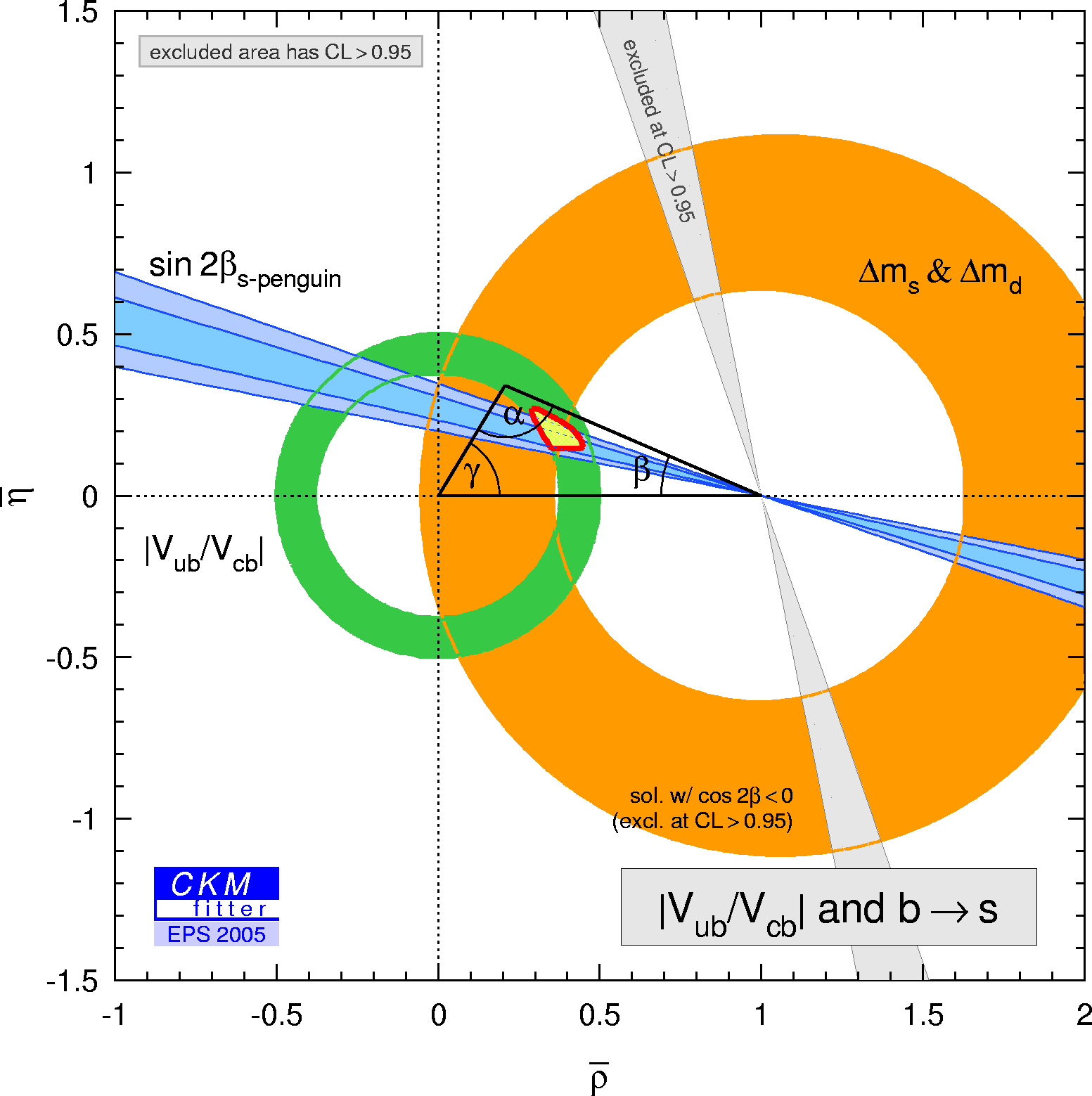eps gif

New physics in B0- B0bar Mixing can be described model-independently by introducing two new parameters measuring the relative strength (rd2) and the relative phase between the B0- B0bar mixing matrix element containing contributions from SM as well as from NP contributions compared to SM contributions only:
 rd2 * exp(i 2*Θd) = -------------------------------
Constraints from |Vcb| + |Vub| , Δmd=Δmd{SM} * rd2 and sin(2β+2Θd) in the (ρ-bar,η-bar) plane.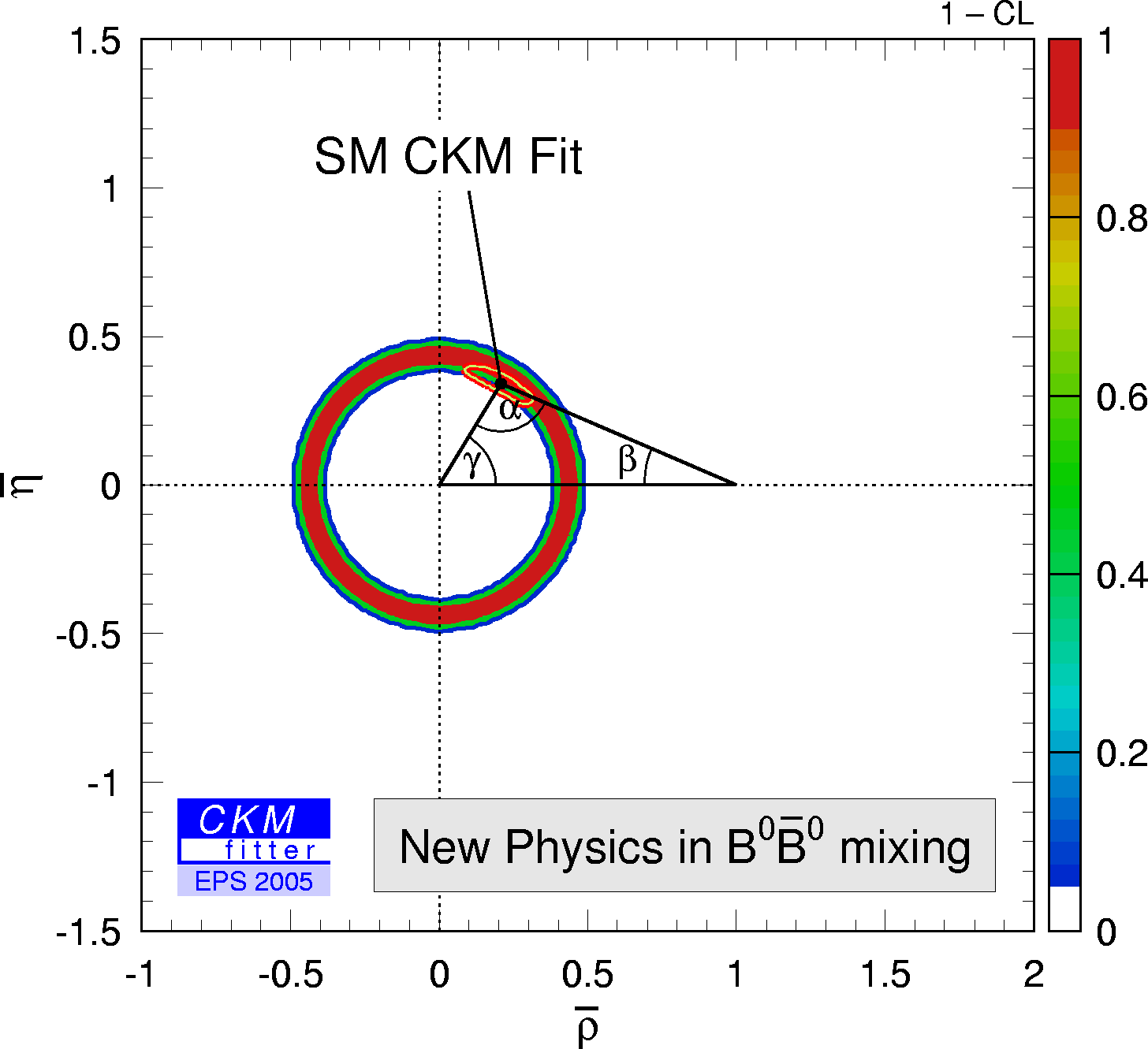eps
gif
Constraints from |Vcb| + |Vub| , Δmd=Δmd{SM} * rd2 and sin(2β+2Θd) in the (2Θd , rd2) plane.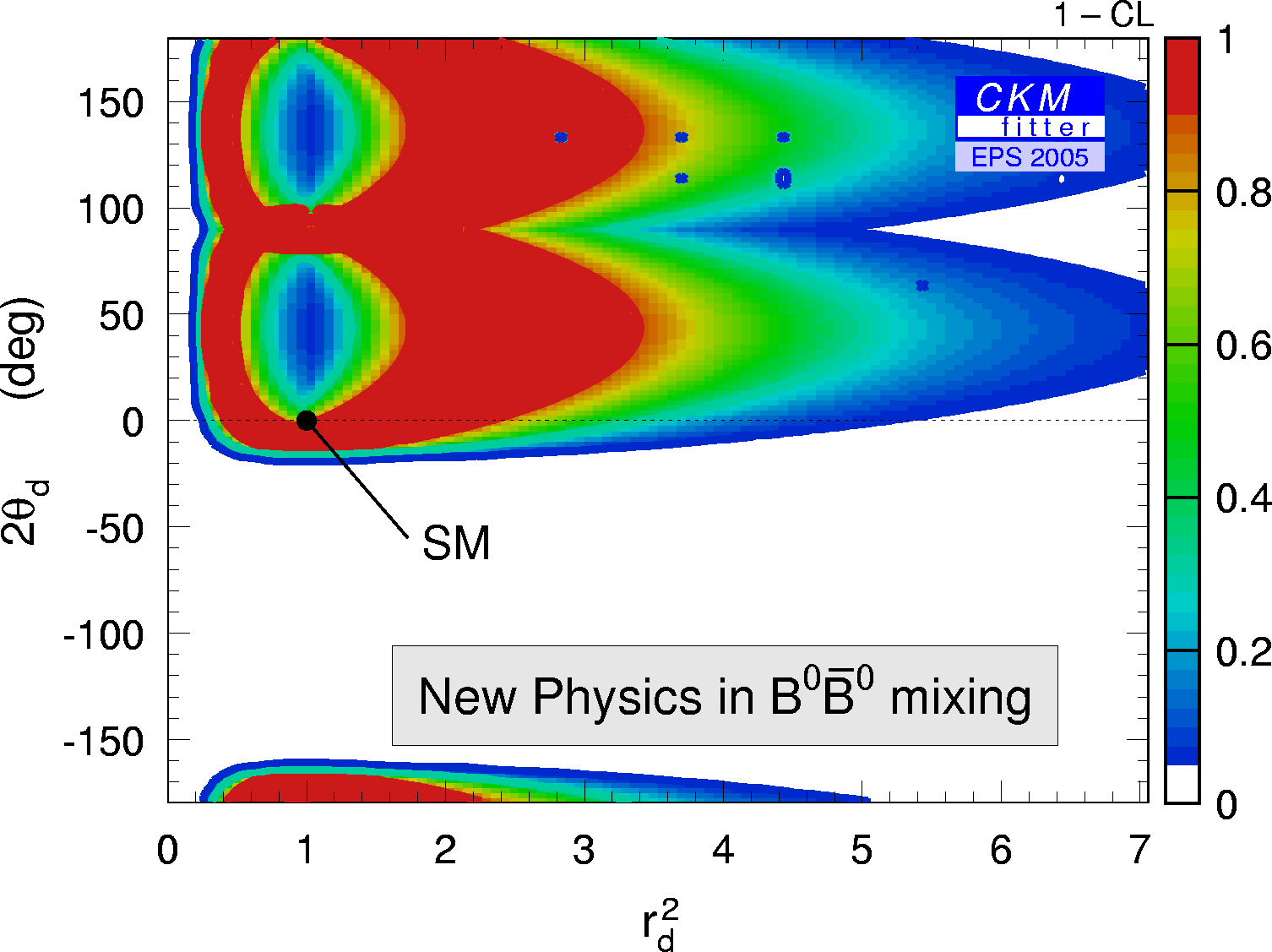eps
gif
Since there are four free parameters in the fit (ρ-bar,η-bar,2*Θd,rd2) but only three constraints depend on those there is no other constraint in the (ρ-bar,η-bar) plane visible but the one coming from |Vub| and |Vcb| alone. As a consequence, the allowed region in the (2*Θd,rd2) plane is large.

When using in addition the following inputs:
cos(2β) > 0 (suggested by data), α (ππ, 3π, ρρ), γ the allowed regions are substantially reduced:
in the (ρ-bar,η-bar) plane.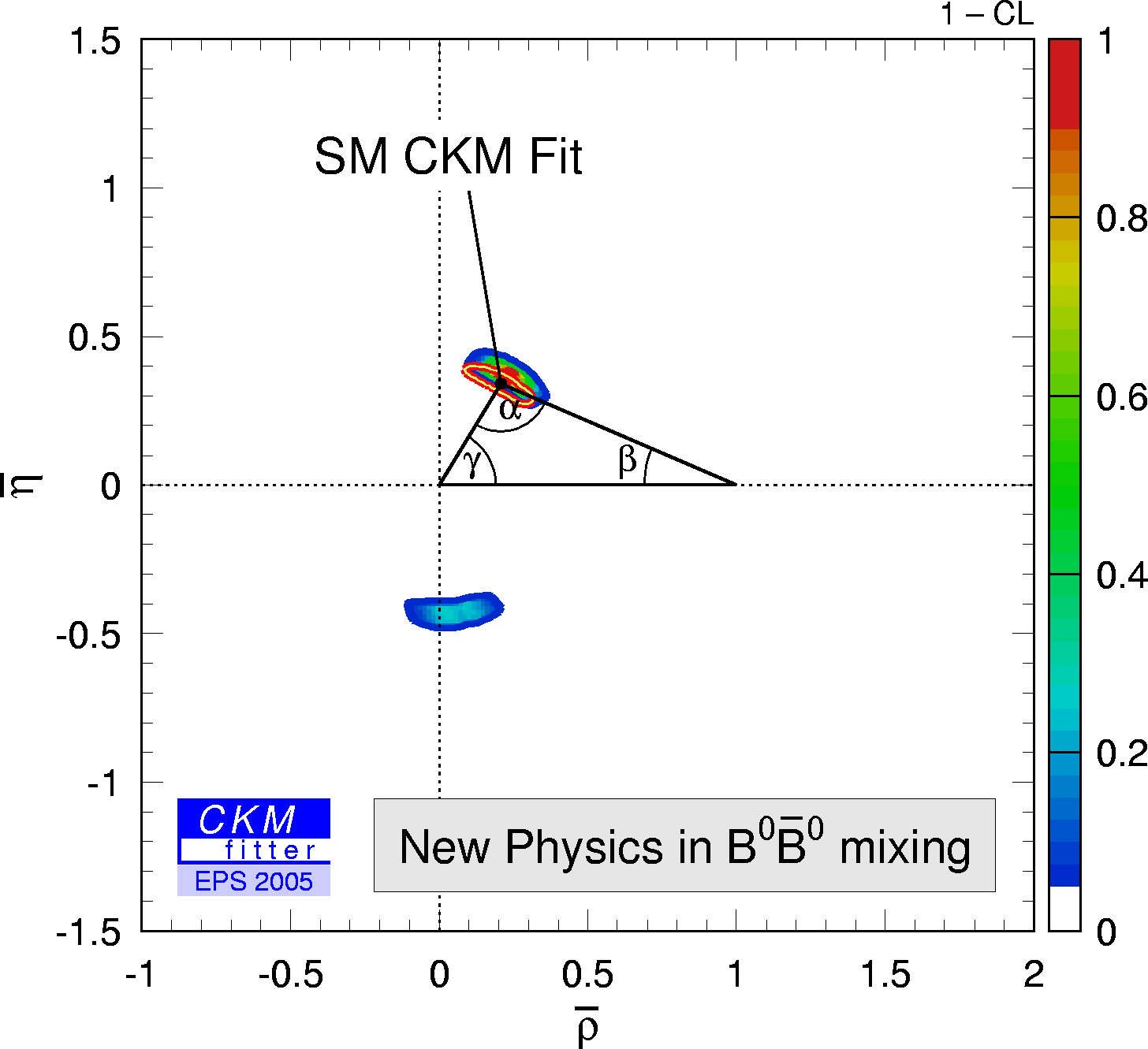eps
gif
in the (2*Θd,rd2) plane.eps
gif
There are two solutions left. The SM solution (2*Θd = 0 ,rd2 = 1) is prefered. If the other solution can be eliminated with more data the possible additional phase from NP could not be very large (<15 degree). The relative strength of NP to SM contributions can still easily be of order 100%.